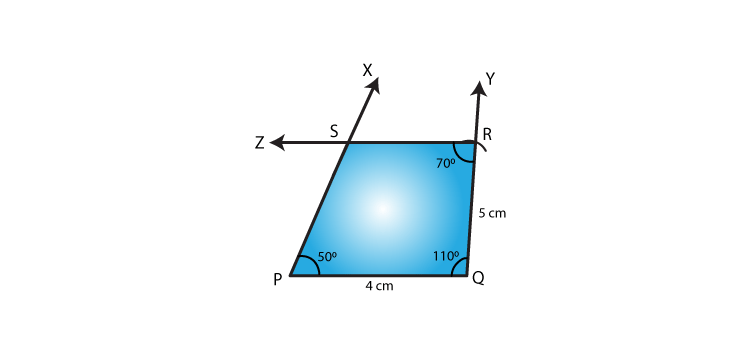# RD Sharma Solutions for Class 8 Maths Chapter 18 Practical Geometry (Constructions)

As the chapter is about Constructions, in Chapter 18 of RD Sharma Class 8 Maths, we shall learn how to construct a quadrilateral with given elements. Students are provided with exercise-wise solutions to help understand the concepts clearly from the exam point of view. The solutions are prepared by the experienced faculty team at BYJU’S, who has explained the concepts in detail which is very helpful for preparing for their board exams. Students can download RD Sharma Class 8 PDF from the links given below.

Chapter 18- Practical Geometry (Constructions) contains five exercises and the RD Sharma Class 8 Solutions present in this page provide solutions to the questions present in each exercise. Now, let us have a look at the concepts discussed in this chapter.

• Constructing a quadrilateral when four sides and one diagonal are given.
• Constructing a quadrilateral when its three sides and the two diagonals are given.
• Constructing a quadrilateral when its four sides and one angle are given.
• Constructing a quadrilateral when its three sides and their included angles are given.
• Constructing a quadrilateral when its three angles and their two included sides are given.

## Download the Pdf of RD Sharma Solutions for Class 8 Maths Chapter 18 Practical Geometry (Constructions)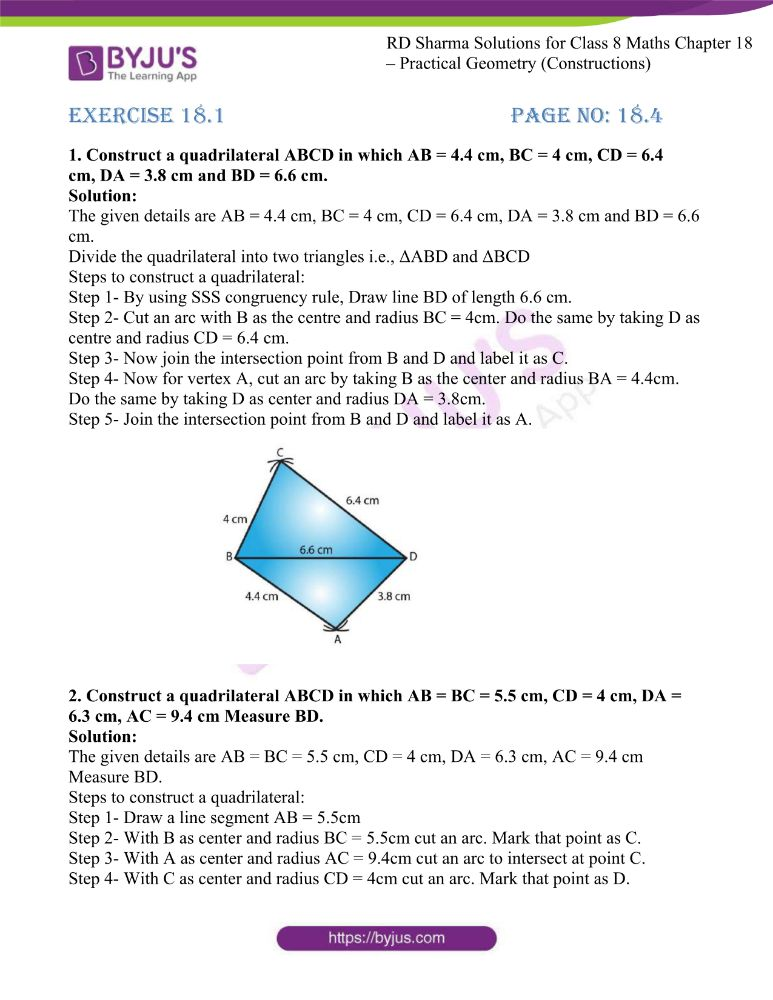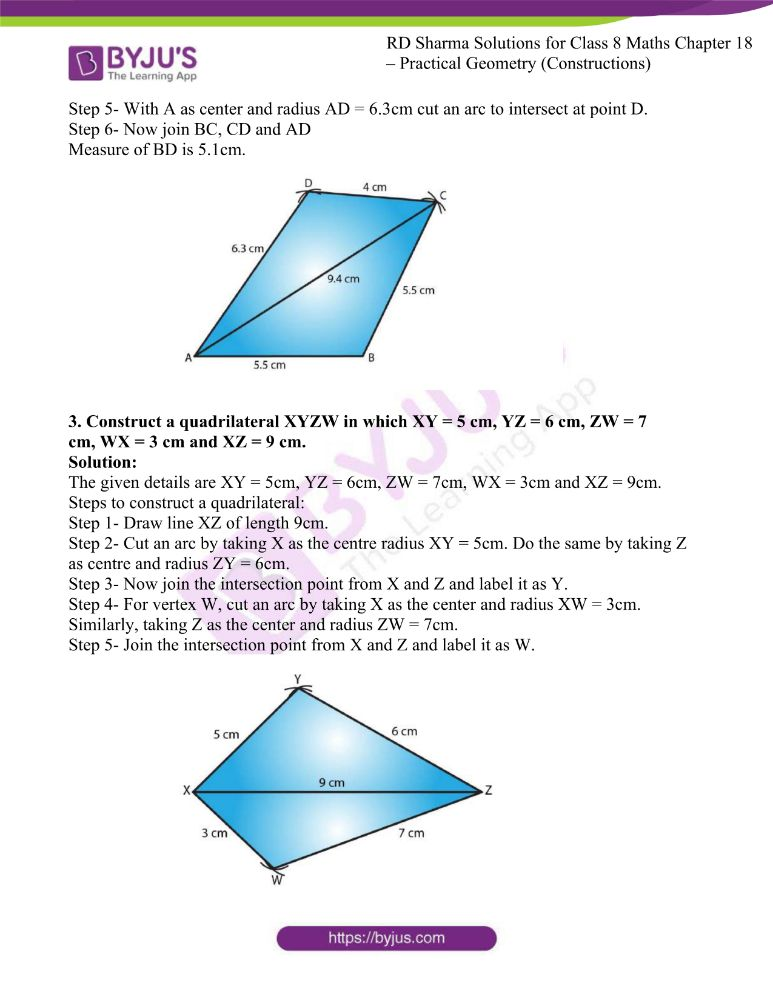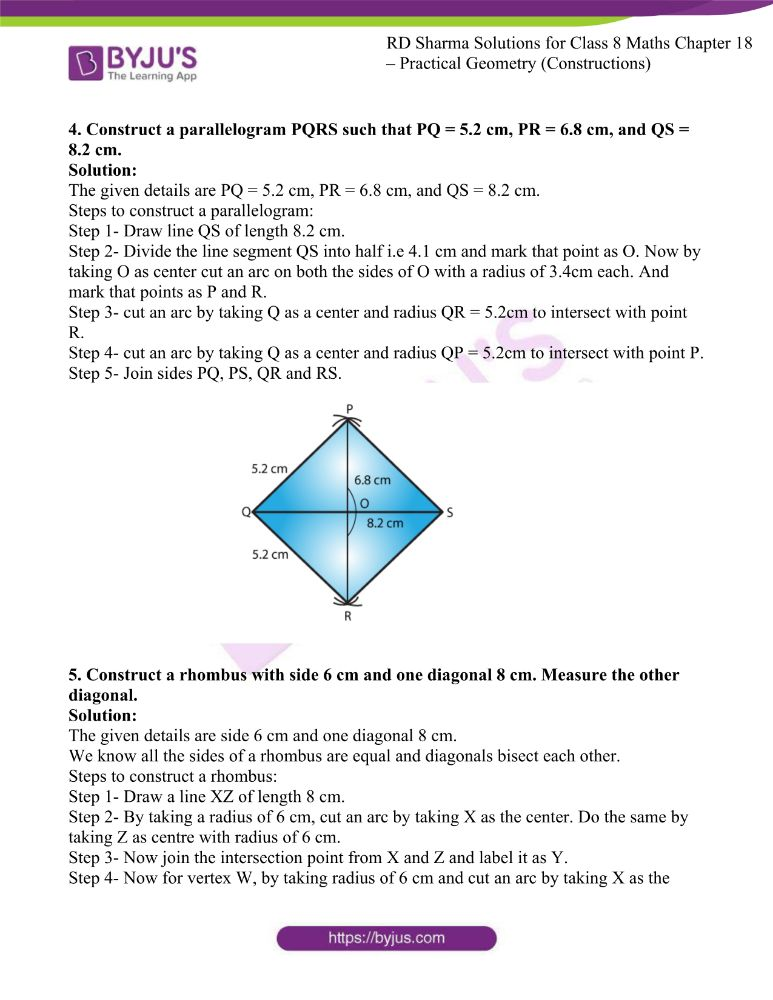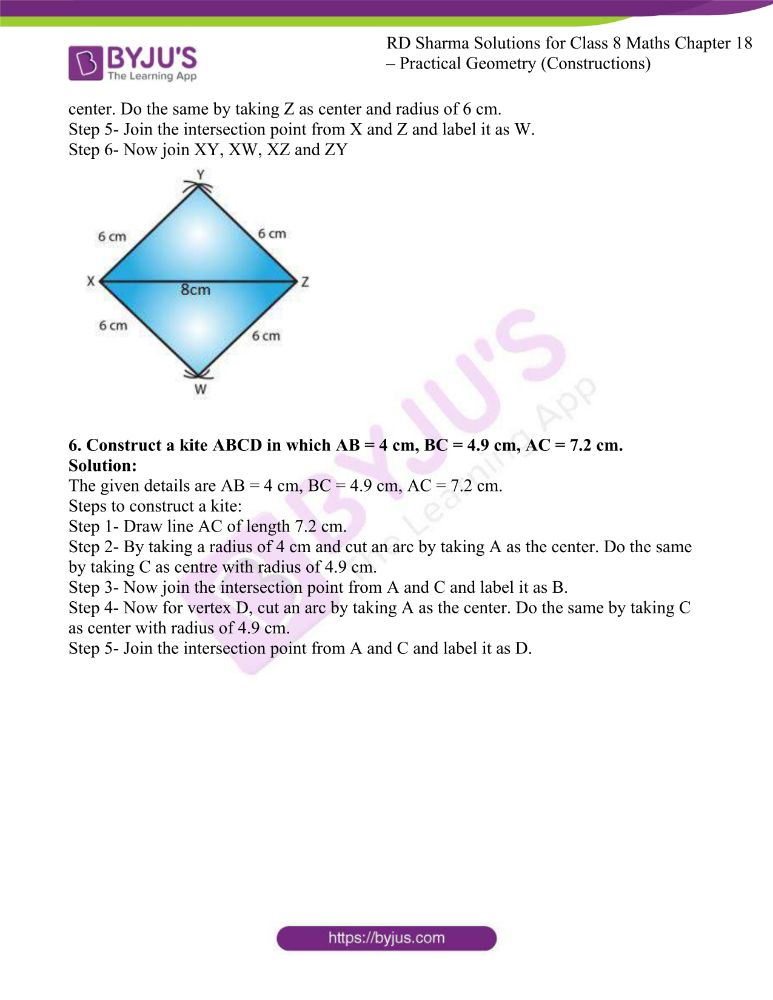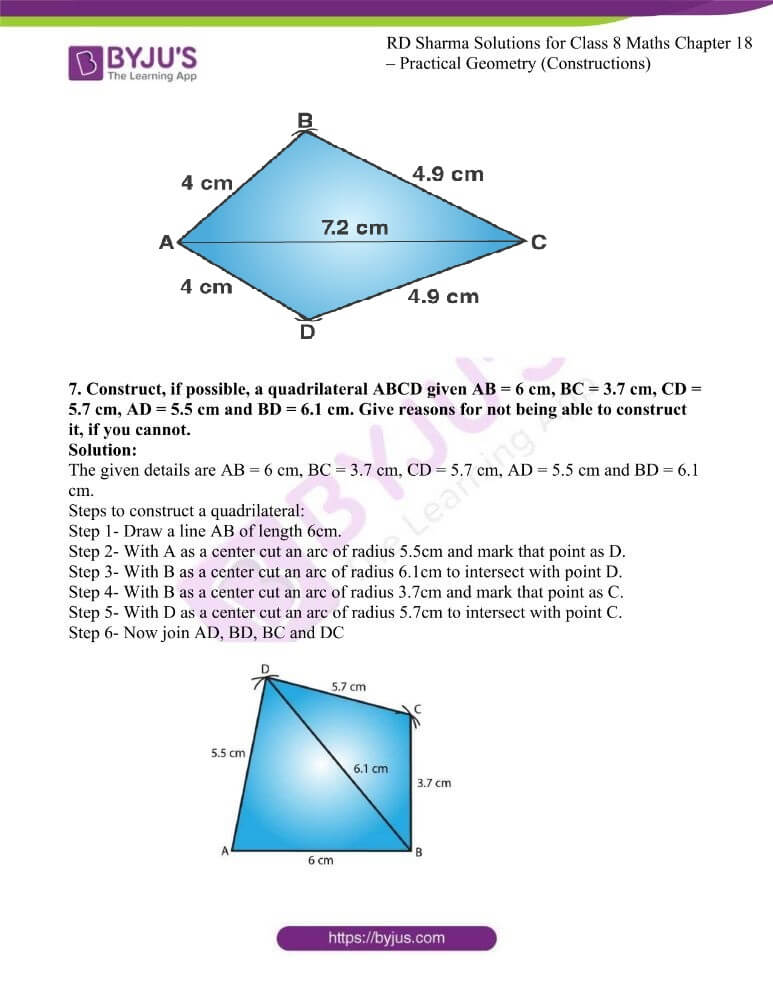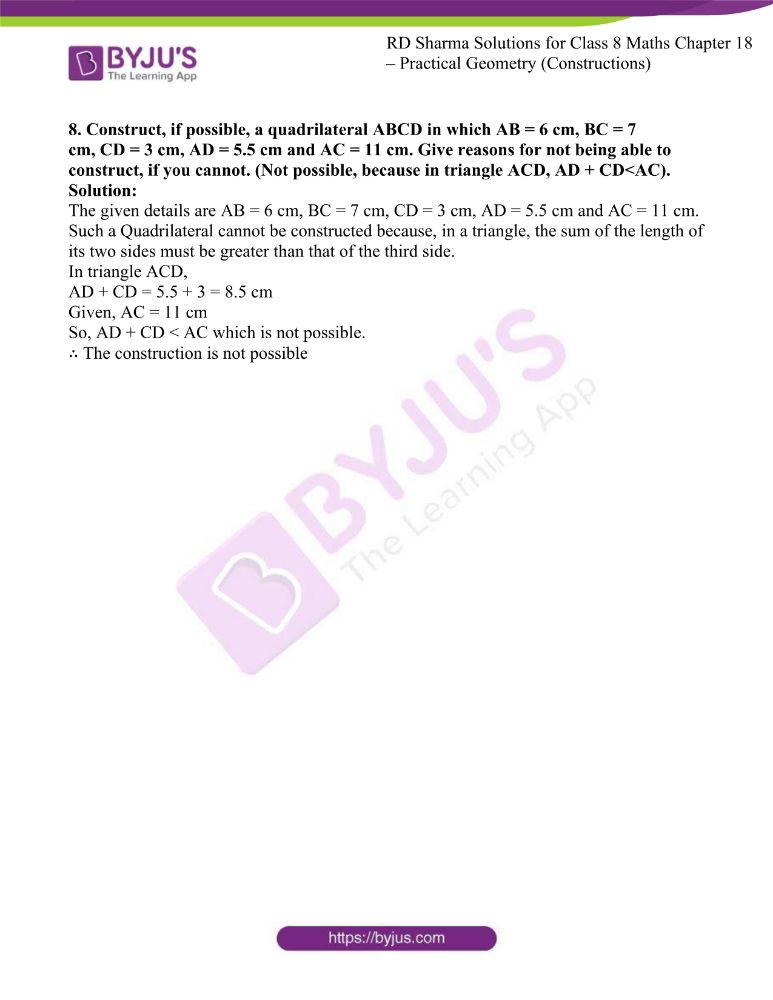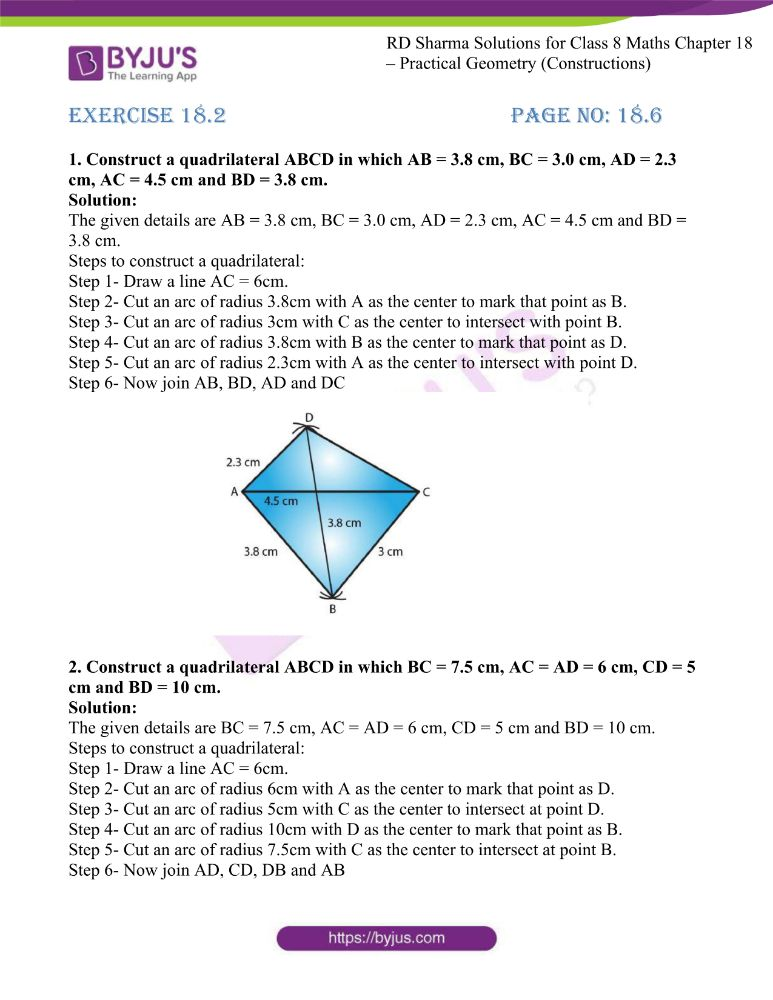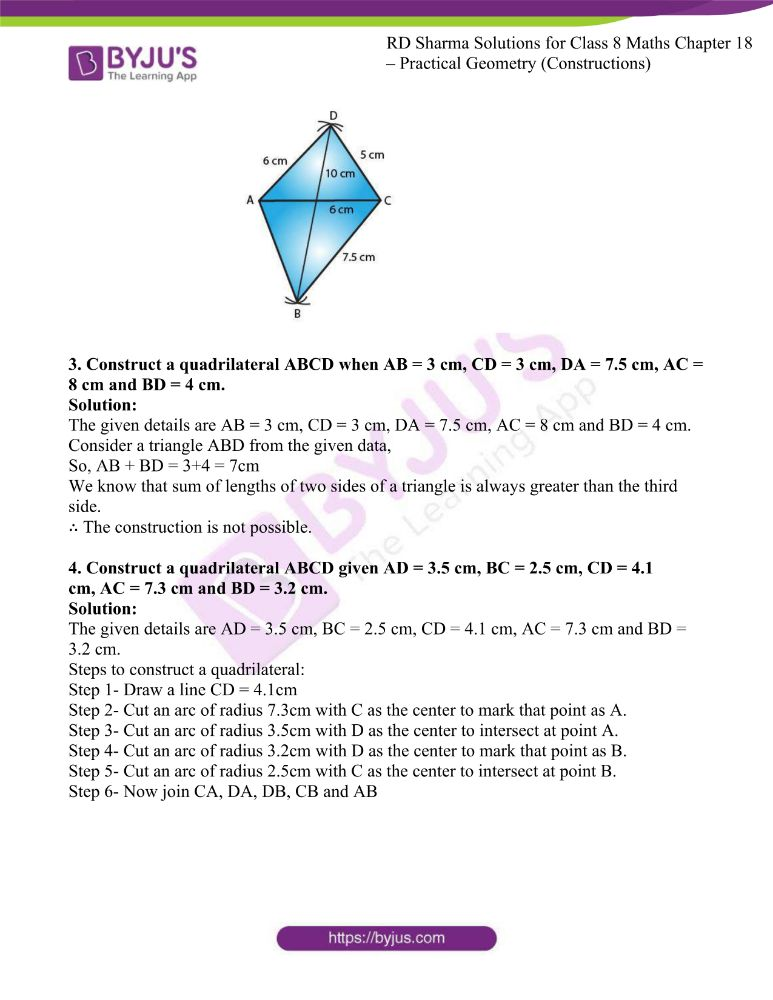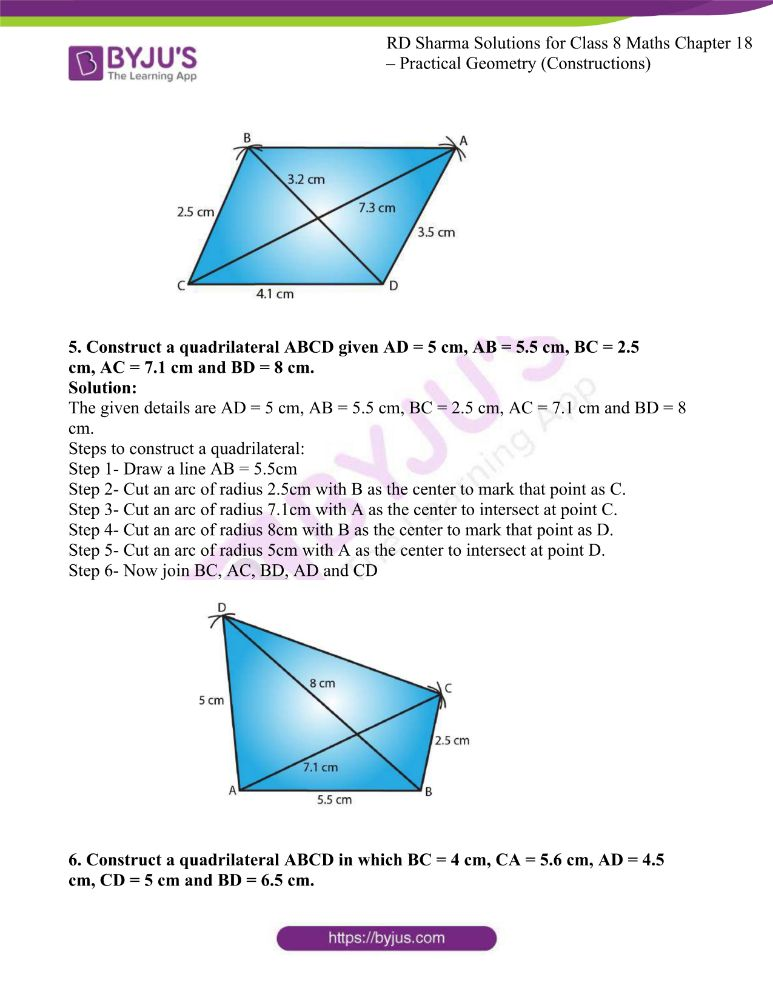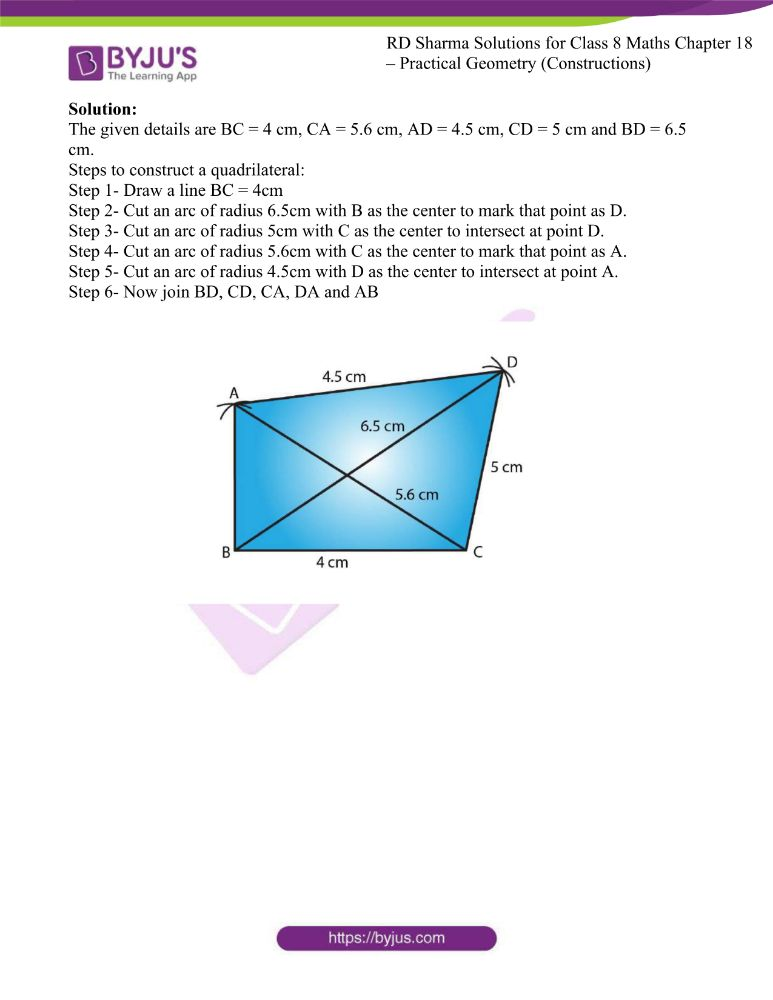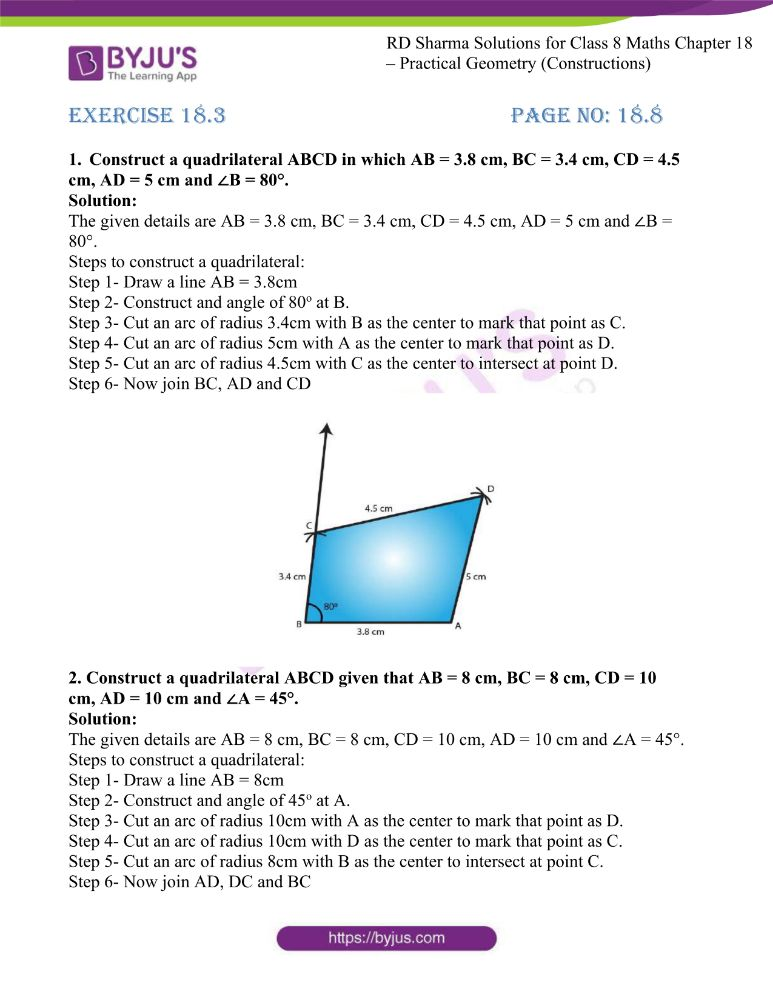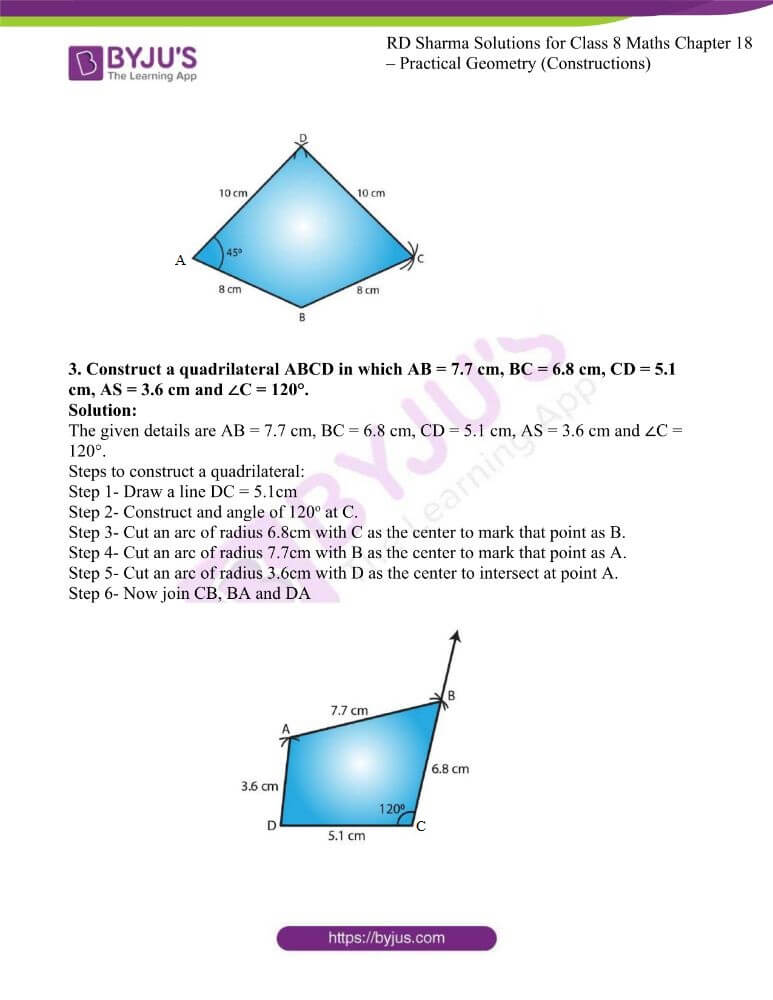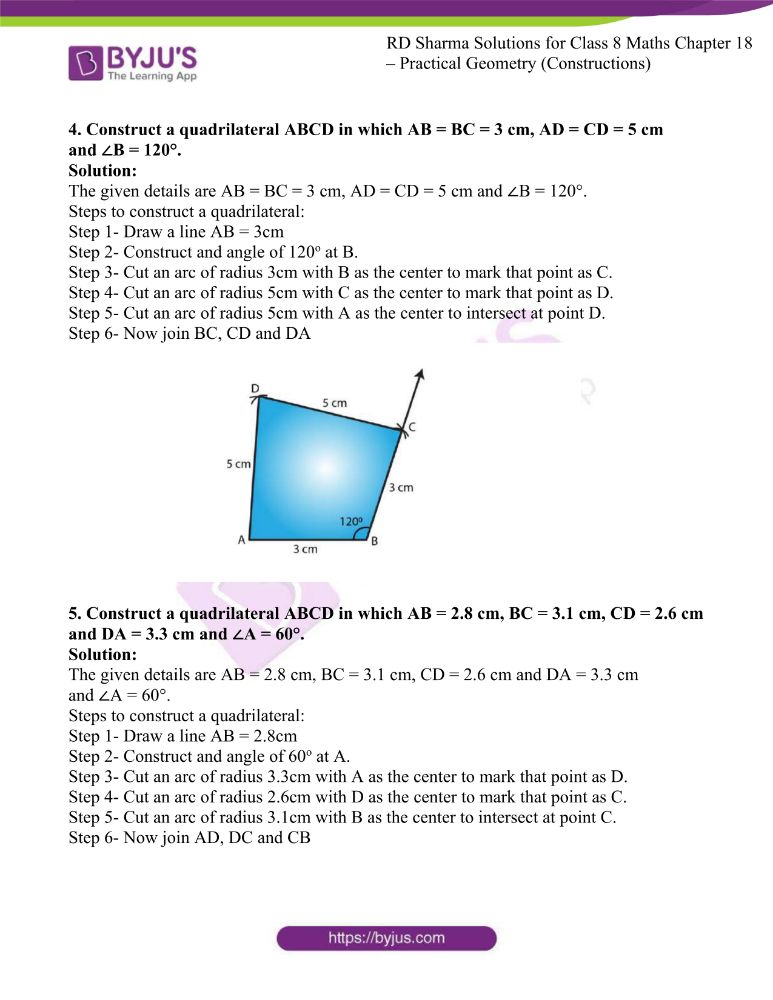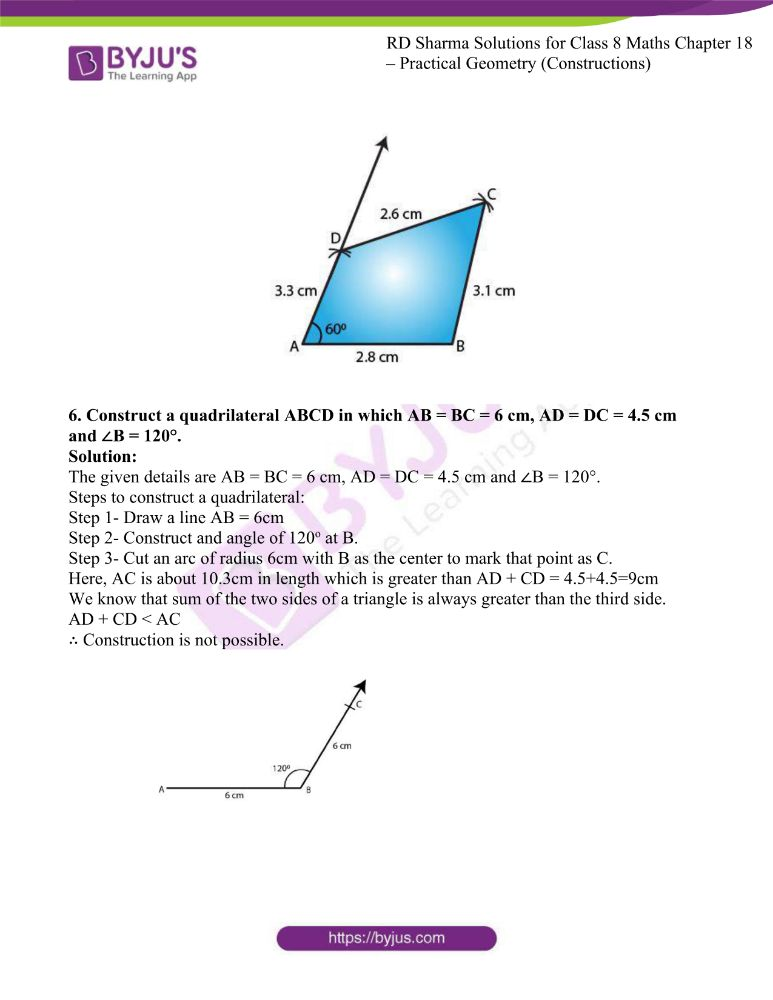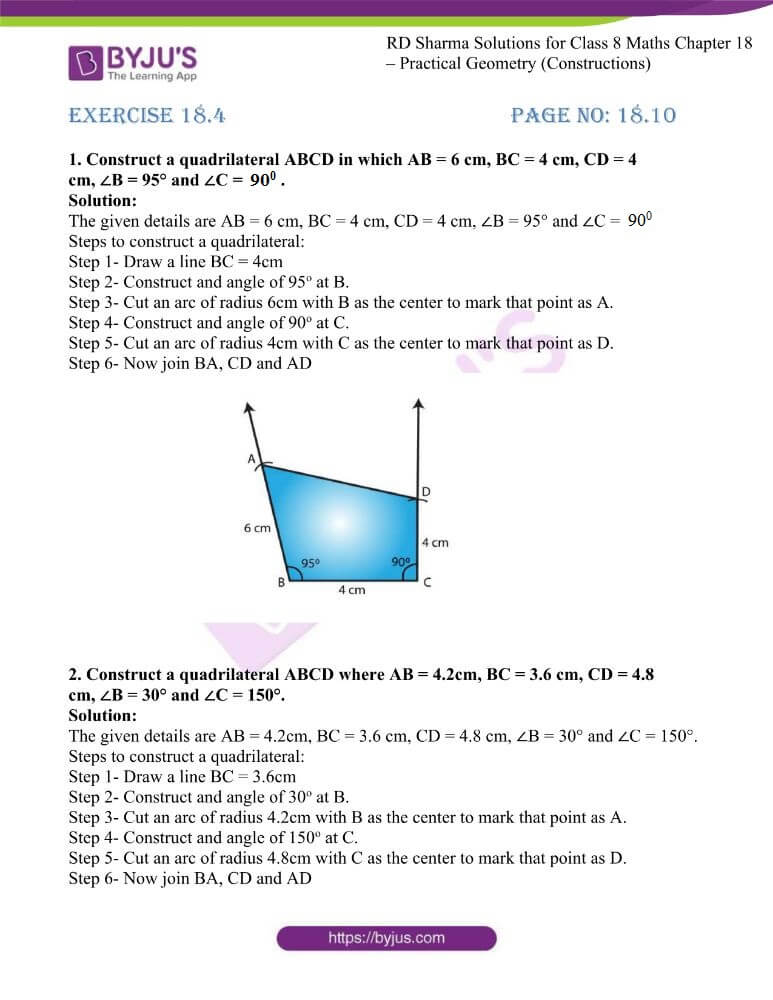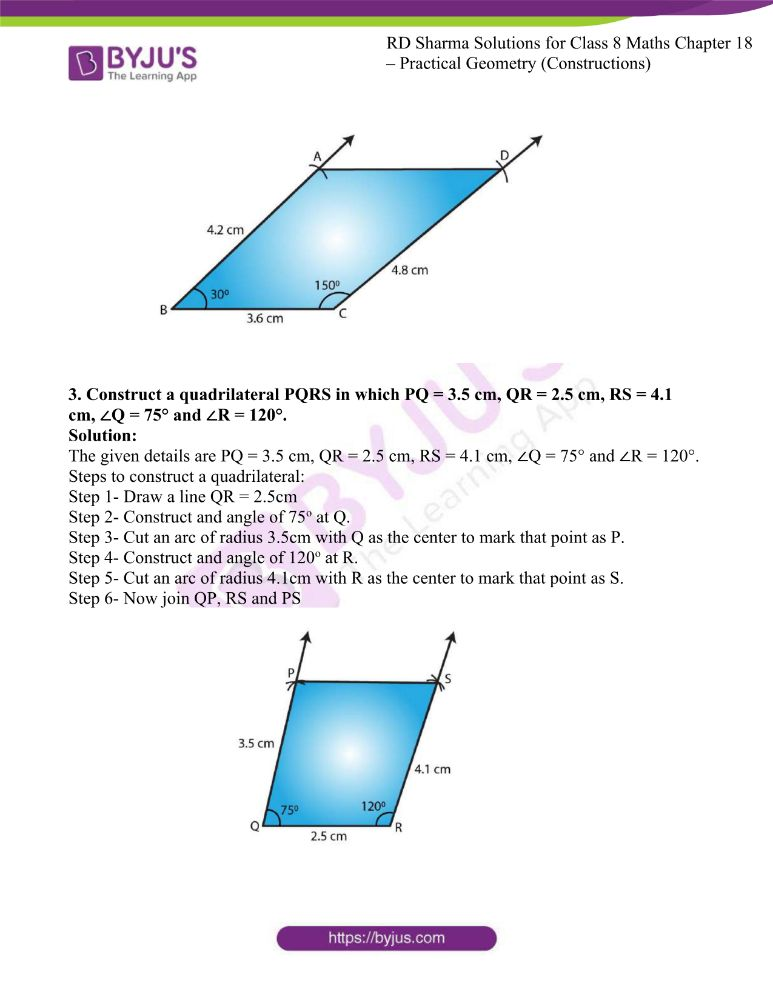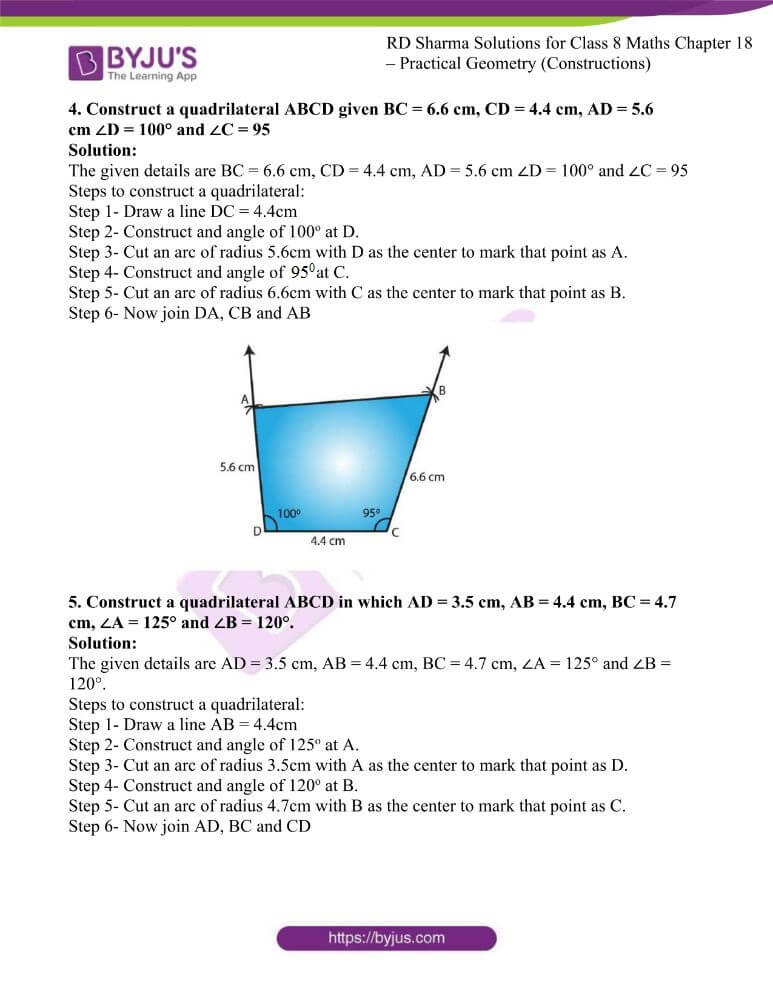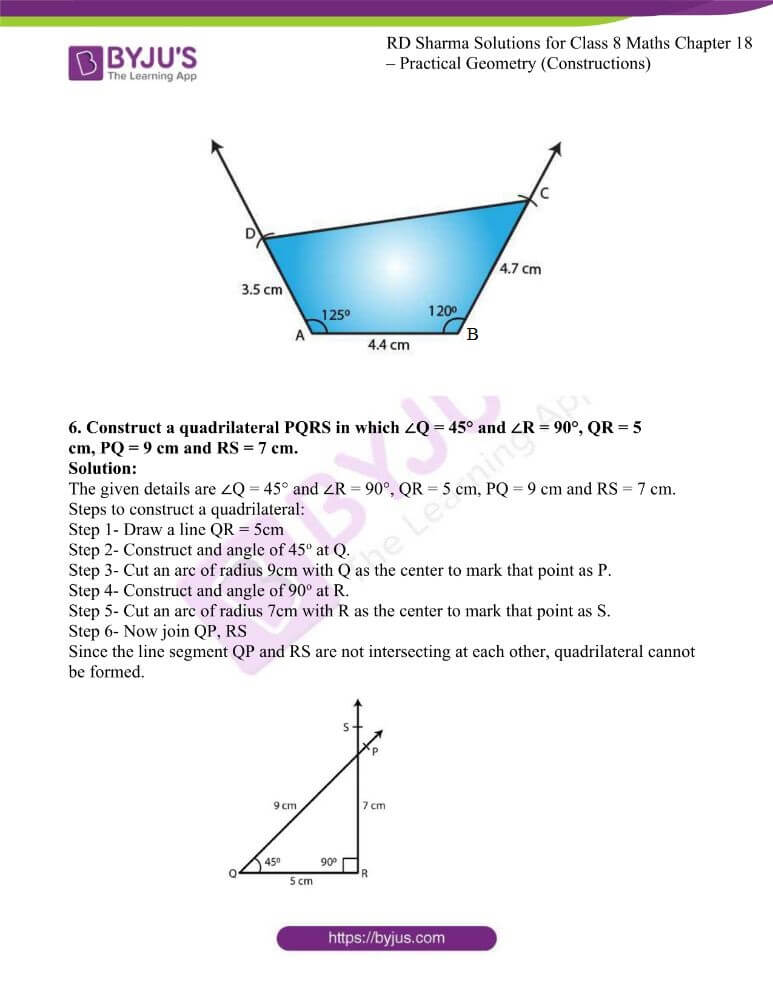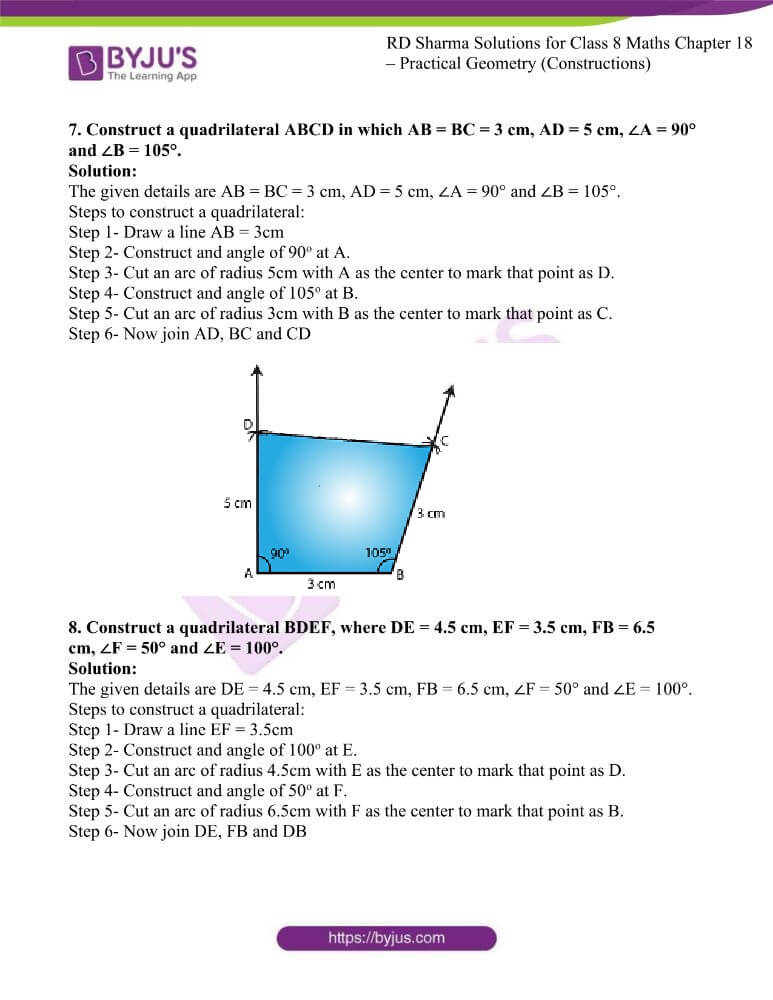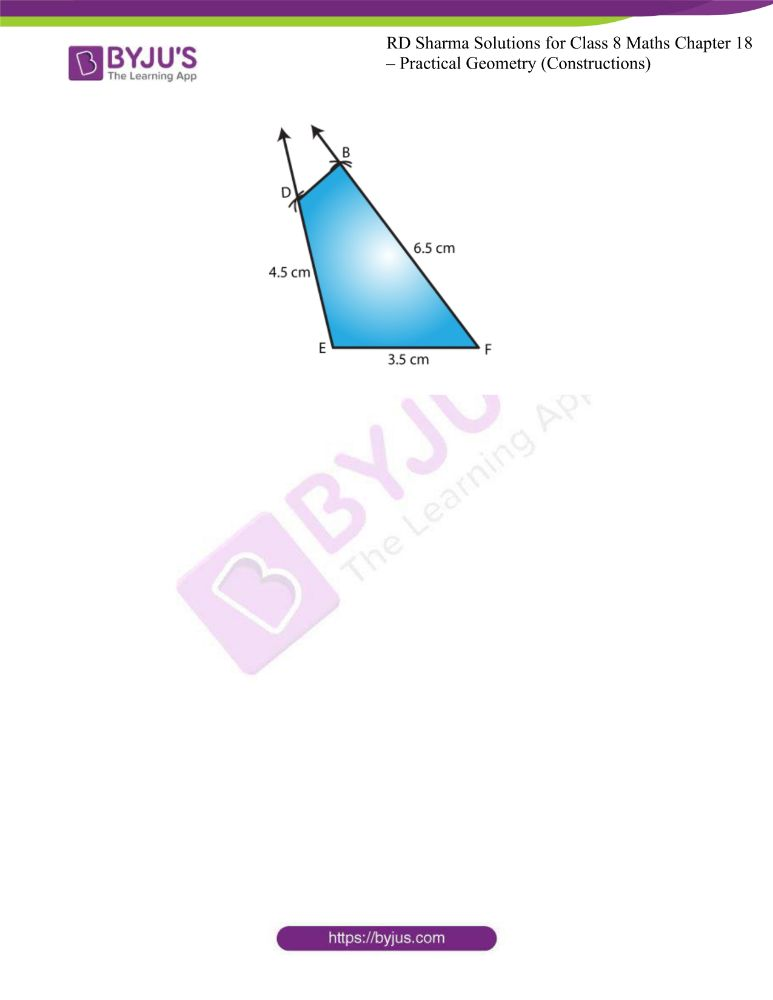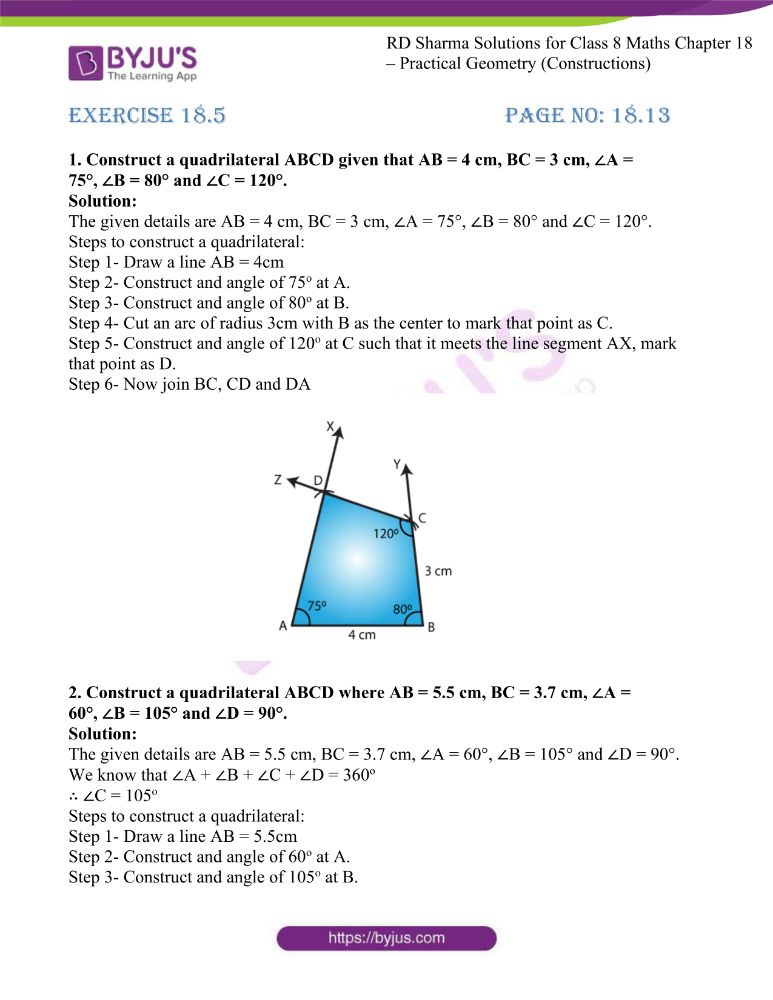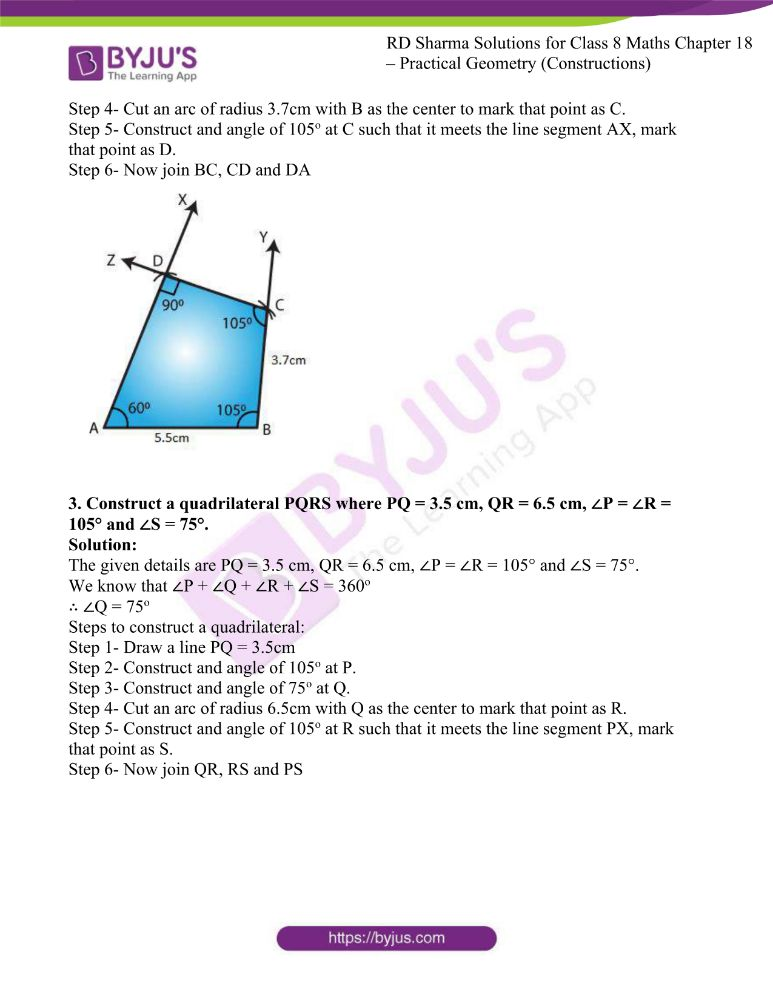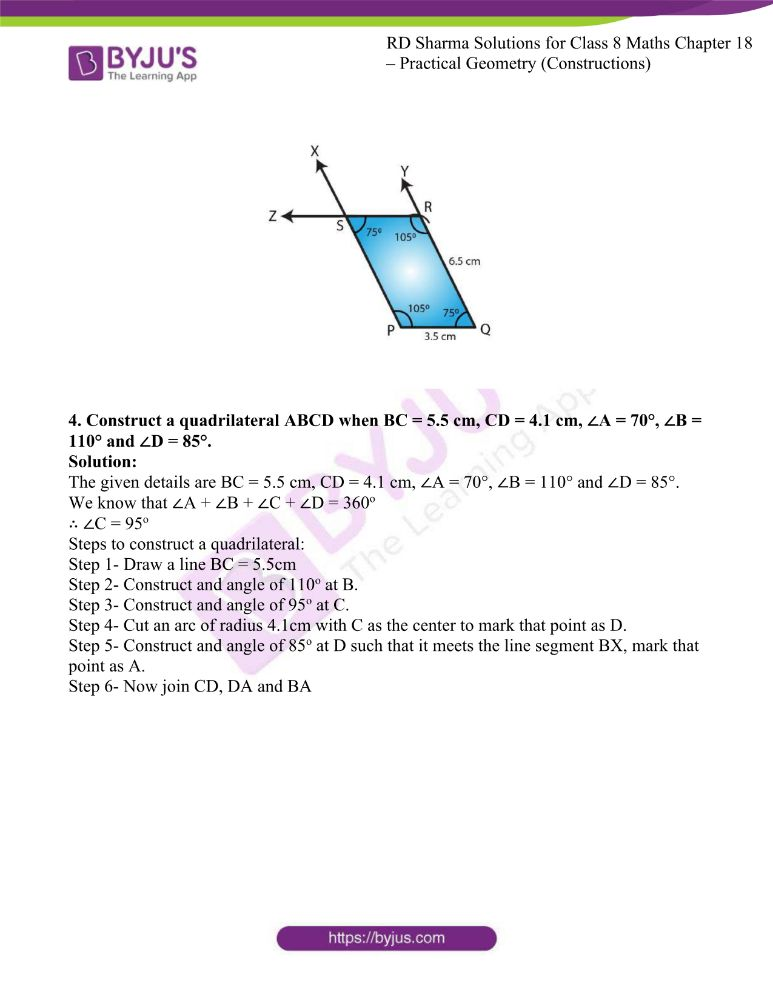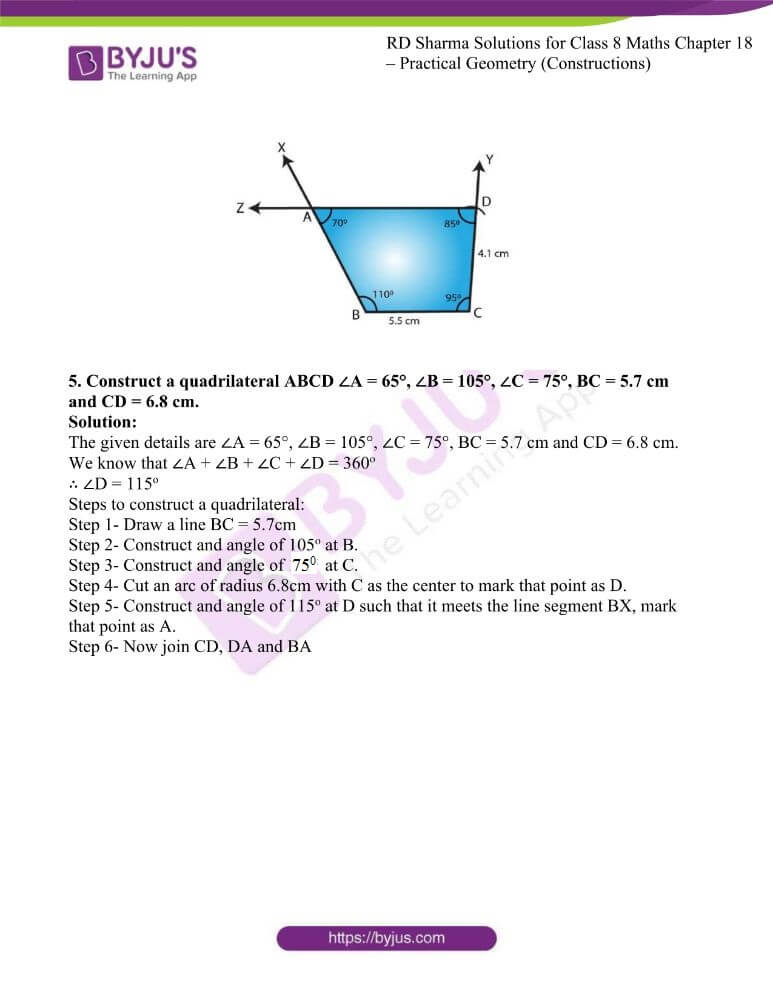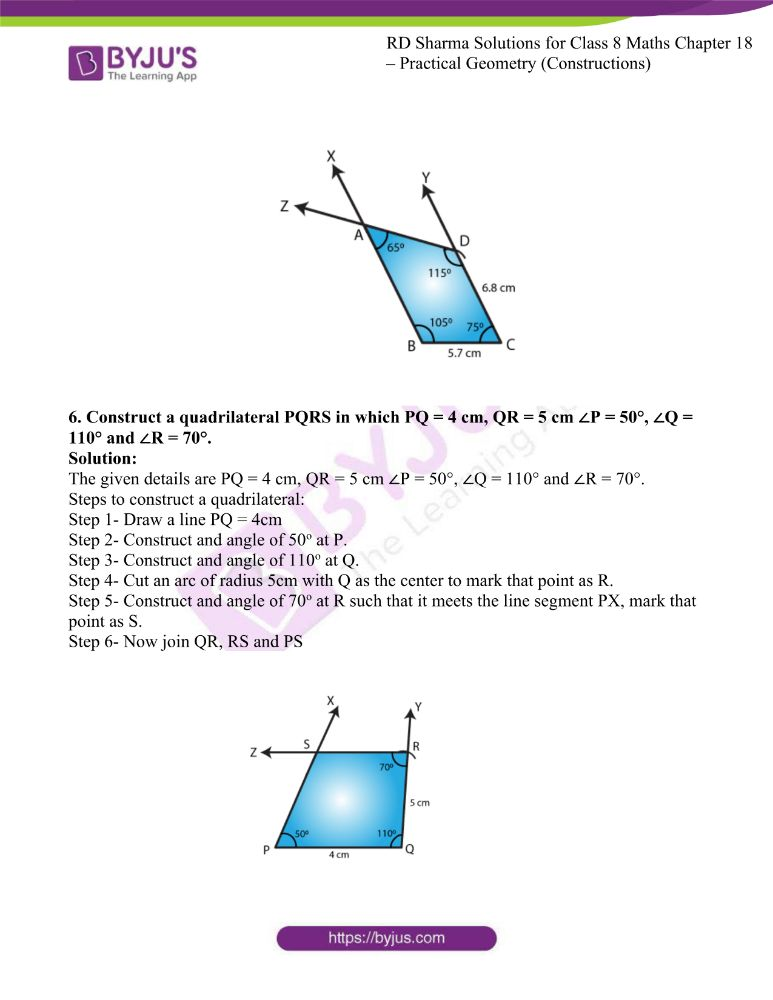### Access answers to Maths RD Sharma Solutions For Class 8 Chapter 18 Practical Geometry (Constructions)

EXERCISE 18.1 PAGE NO: 18.4

1. Construct a quadrilateral ABCD in which AB = 4.4 cm, BC = 4 cm, CD = 6.4 cm, DA = 3.8 cm and BD = 6.6 cm.

Solution:

The given details are AB = 4.4 cm, BC = 4 cm, CD = 6.4 cm, DA = 3.8 cm and BD = 6.6 cm.

Divide the quadrilateral into two triangles i.e., ΔABD and ΔBCD

Step 1- By using SSS congruency rule, Draw line BD of length 6.6 cm.

Step 2- Cut an arc with B as the centre and radius BC = 4cm. Do the same by taking D as centre and radius CD = 6.4 cm.

Step 3- Now join the intersection point from B and D and label it as C.

Step 4- Now for vertex A, cut an arc by taking B as the center and radius BA = 4.4cm. Do the same by taking D as center and radius DA = 3.8cm.

Step 5- Join the intersection point from B and D and label it as A.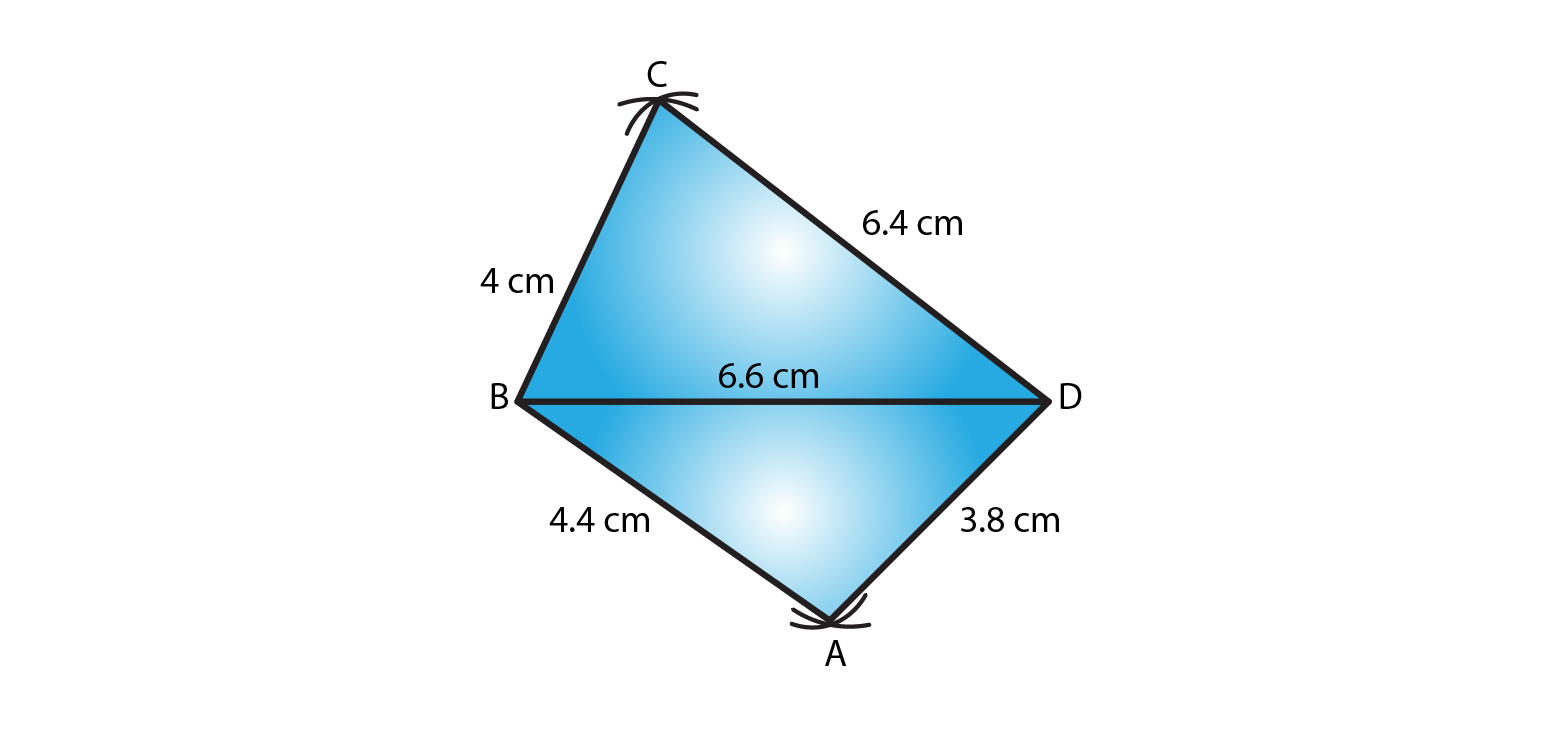2. Construct a quadrilateral ABCD in which AB = BC = 5.5 cm, CD = 4 cm, DA = 6.3 cm, AC = 9.4 cm Measure BD.

Solution:

The given details are AB = BC = 5.5 cm, CD = 4 cm, DA = 6.3 cm, AC = 9.4 cm Measure BD.

Step 1- Draw a line segment AB = 5.5cm

Step 2- With B as center and radius BC = 5.5cm cut an arc. Mark that point as C.

Step 3- With A as center and radius AC = 9.4cm cut an arc to intersect at point C.

Step 4- With C as center and radius CD = 4cm cut an arc. Mark that point as D.

Step 5- With A as center and radius AD = 6.3cm cut an arc to intersect at point D.

Step 6- Now join BC, CD and AD

Measure of BD is 5.1cm.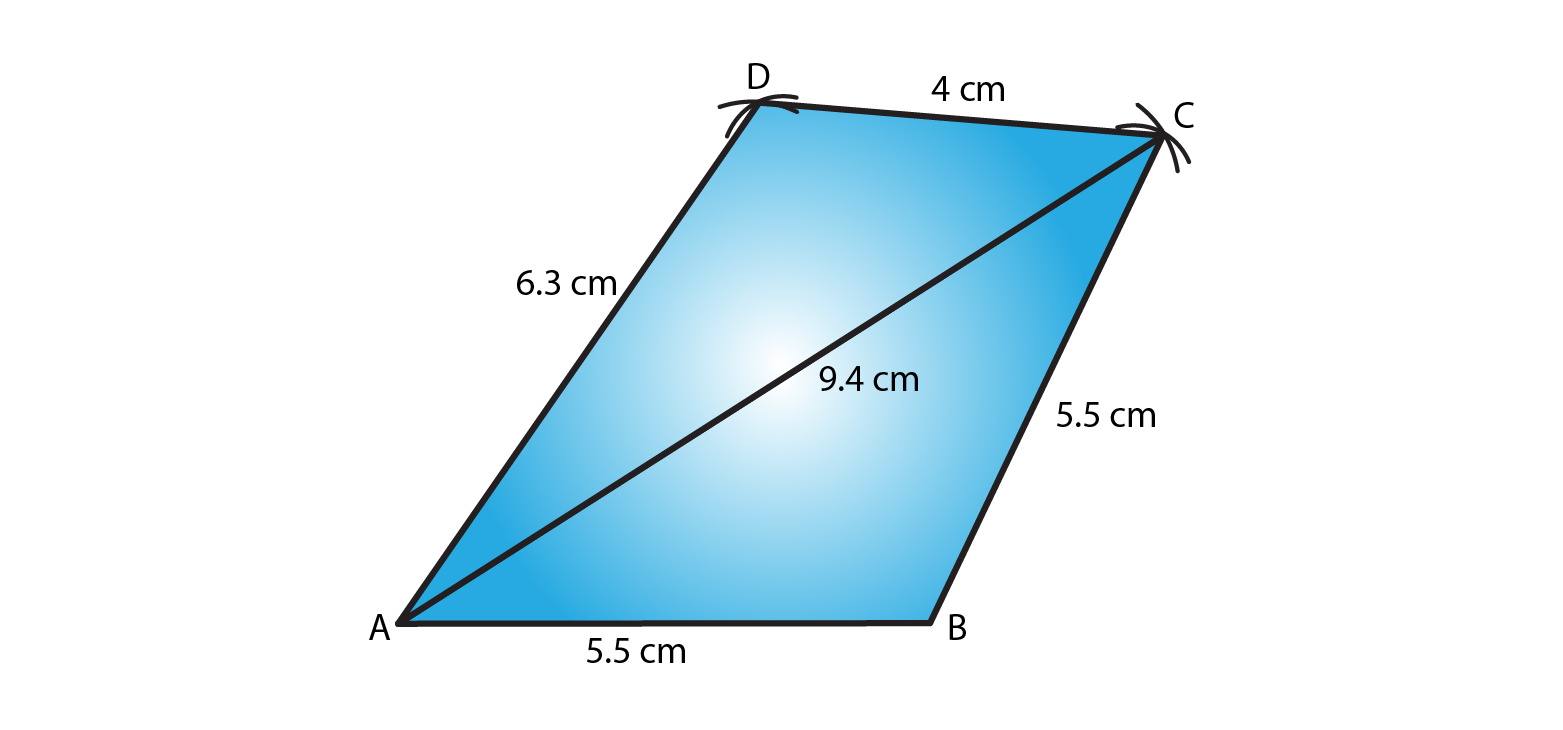3. Construct a quadrilateral XYZW in which XY = 5 cm, YZ = 6 cm, ZW = 7 cm, WX = 3 cm and XZ = 9 cm.

Solution:

The given details are XY = 5cm, YZ = 6cm, ZW = 7cm, WX = 3cm and XZ = 9cm.

Step 1- Draw line XZ of length 9cm.

Step 2- Cut an arc by taking X as the centre radius XY = 5cm. Do the same by taking Z as centre and radius ZY = 6cm.

Step 3- Now join the intersection point from X and Z and label it as Y.

Step 4- For vertex W, cut an arc by taking X as the center and radius XW = 3cm. Similarly, taking Z as the center and radius ZW = 7cm.

Step 5- Join the intersection point from X and Z and label it as W.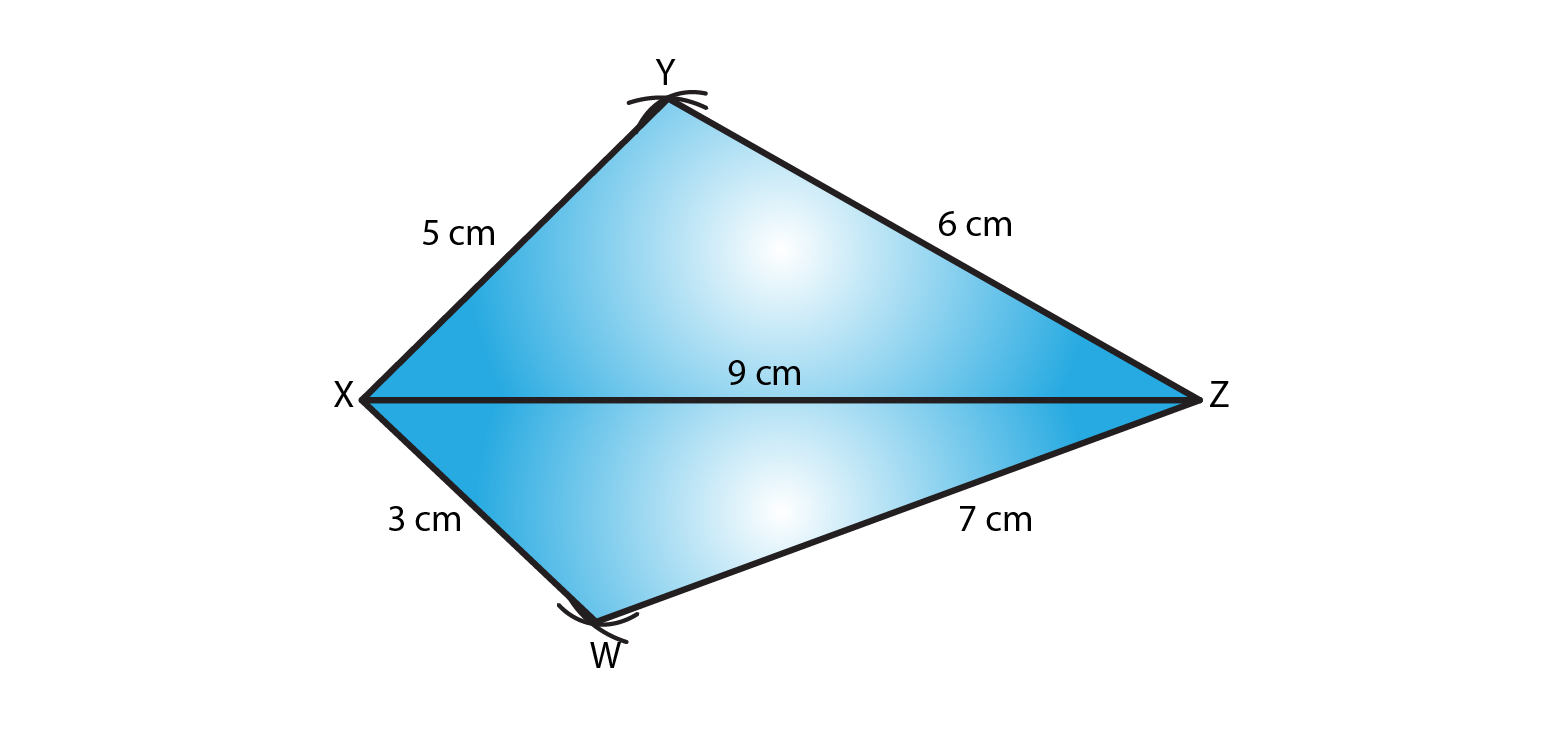4. Construct a parallelogram PQRS such that PQ = 5.2 cm, PR = 6.8 cm, and QS = 8.2 cm.

Solution:

The given details are PQ = 5.2 cm, PR = 6.8 cm, and QS = 8.2 cm.

Steps to construct a parallelogram:

Step 1- Draw line QS of length 8.2 cm.

Step 2- Divide the line segment QS into half i.e 4.1 cm and mark that point as O. Now by taking O as center cut an arc on both the sides of O with a radius of 3.4cm each. And mark that points as P and R.

Step 3- cut an arc by taking Q as a center and radius QR = 5.2cm to intersect with point R.

Step 4- cut an arc by taking Q as a center and radius QP = 5.2cm to intersect with point P.

Step 5- Join sides PQ, PS, QR and RS.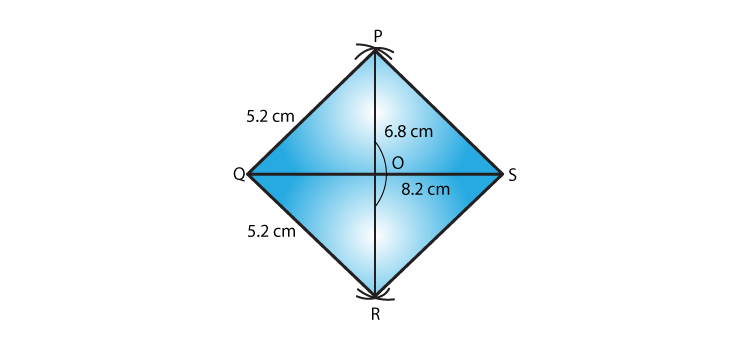5. Construct a rhombus with side 6 cm and one diagonal 8 cm. Measure the other diagonal.

Solution:

The given details are side 6 cm and one diagonal 8 cm.

We know all the sides of a rhombus are equal and diagonals bisect each other.

Steps to construct a rhombus:

Step 1- Draw a line XZ of length 8 cm.

Step 2- By taking a radius of 6 cm, cut an arc by taking X as the center. Do the same by taking Z as centre with radius of 6 cm.

Step 3- Now join the intersection point from X and Z and label it as Y.

Step 4- Now for vertex W, by taking radius of 6 cm and cut an arc by taking X as the center. Do the same by taking Z as center and radius of 6 cm.

Step 5- Join the intersection point from X and Z and label it as W.

Step 6- Now join XY, XW, XZ and ZY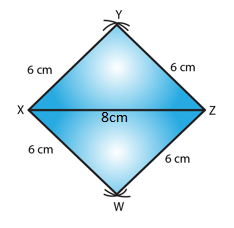6. Construct a kite ABCD in which AB = 4 cm, BC = 4.9 cm, AC = 7.2 cm.

Solution:

The given details are AB = 4 cm, BC = 4.9 cm, AC = 7.2 cm.

Steps to construct a kite:

Step 1- Draw line AC of length 7.2 cm.

Step 2- By taking a radius of 4 cm and cut an arc by taking A as the center. Do the same by taking C as centre with radius of 4.9 cm.

Step 3- Now join the intersection point from A and C and label it as B.

Step 4- Now for vertex D, cut an arc by taking A as the center. Do the same by taking C as center with radius of 4.9 cm.

Step 5- Join the intersection point from A and C and label it as D.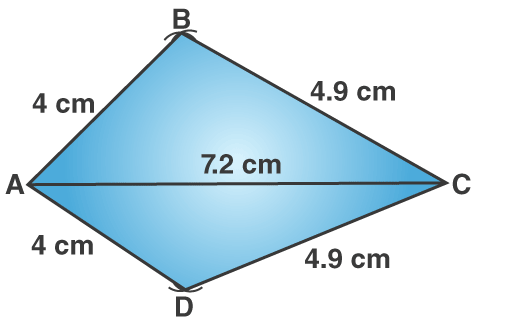7. Construct, if possible, a quadrilateral ABCD given AB = 6 cm, BC = 3.7 cm, CD = 5.7 cm, AD = 5.5 cm and BD = 6.1 cm. Give reasons for not being able to construct it, if you cannot.

Solution:

The given details are AB = 6 cm, BC = 3.7 cm, CD = 5.7 cm, AD = 5.5 cm and BD = 6.1 cm.

Step 1- Draw a line AB of length 6cm.

Step 2- With A as a center cut an arc of radius 5.5cm and mark that point as D.

Step 3- With B as a center cut an arc of radius 6.1cm to intersect with point D.

Step 4- With B as a center cut an arc of radius 3.7cm and mark that point as C.

Step 5- With D as a center cut an arc of radius 5.7cm to intersect with point C.

Step 6- Now join AD, BD, BC and DC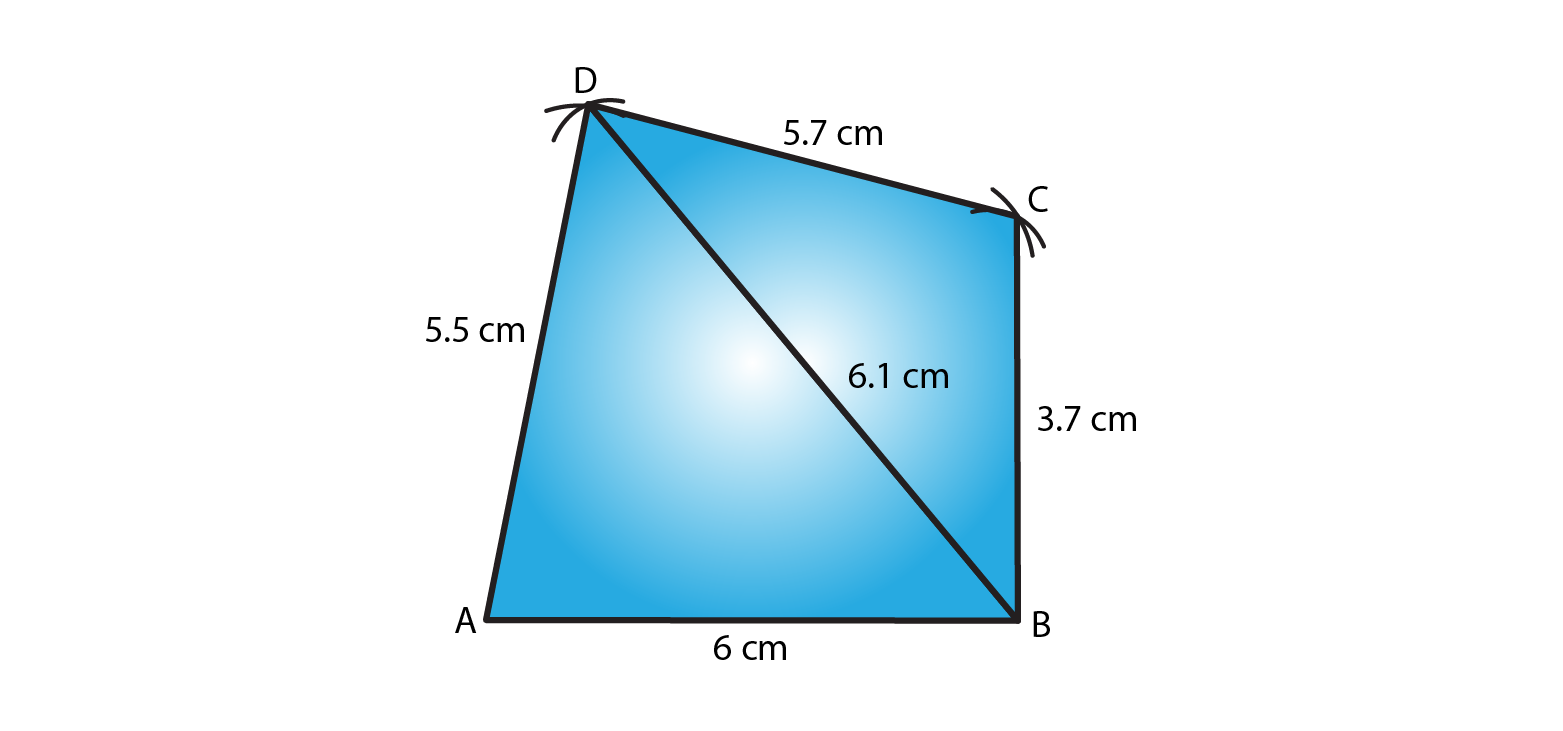8. Construct, if possible, a quadrilateral ABCD in which AB = 6 cm, BC = 7 cm, CD = 3 cm, AD = 5.5 cm and AC = 11 cm. Give reasons for not being able to construct, if you cannot. (Not possible, because in triangle ACD, AD + CD<AC).

Solution:

The given details are AB = 6 cm, BC = 7 cm, CD = 3 cm, AD = 5.5 cm and AC = 11 cm.

Such a Quadrilateral cannot be constructed because, in a triangle, the sum of the length of its two sides must be greater than that of the third side.

In triangle ACD,

AD + CD = 5.5 + 3 = 8.5 cm

Given, AC = 11 cm

So, AD + CD < AC which is not possible.

∴ The construction is not possible

EXERCISE 18.2 PAGE NO: 18.6

1. Construct a quadrilateral ABCD in which AB = 3.8 cm, BC = 3.0 cm, AD = 2.3 cm, AC = 4.5 cm and BD = 3.8 cm.

Solution:

The given details are AB = 3.8 cm, BC = 3.0 cm, AD = 2.3 cm, AC = 4.5 cm and BD = 3.8 cm.

Step 1- Draw a line AC = 6cm.

Step 2- Cut an arc of radius 3.8cm with A as the center to mark that point as B.

Step 3- Cut an arc of radius 3cm with C as the center to intersect with point B.

Step 4- Cut an arc of radius 3.8cm with B as the center to mark that point as D.

Step 5- Cut an arc of radius 2.3cm with A as the center to intersect with point D.

Step 6- Now join AB, BD, AD and DC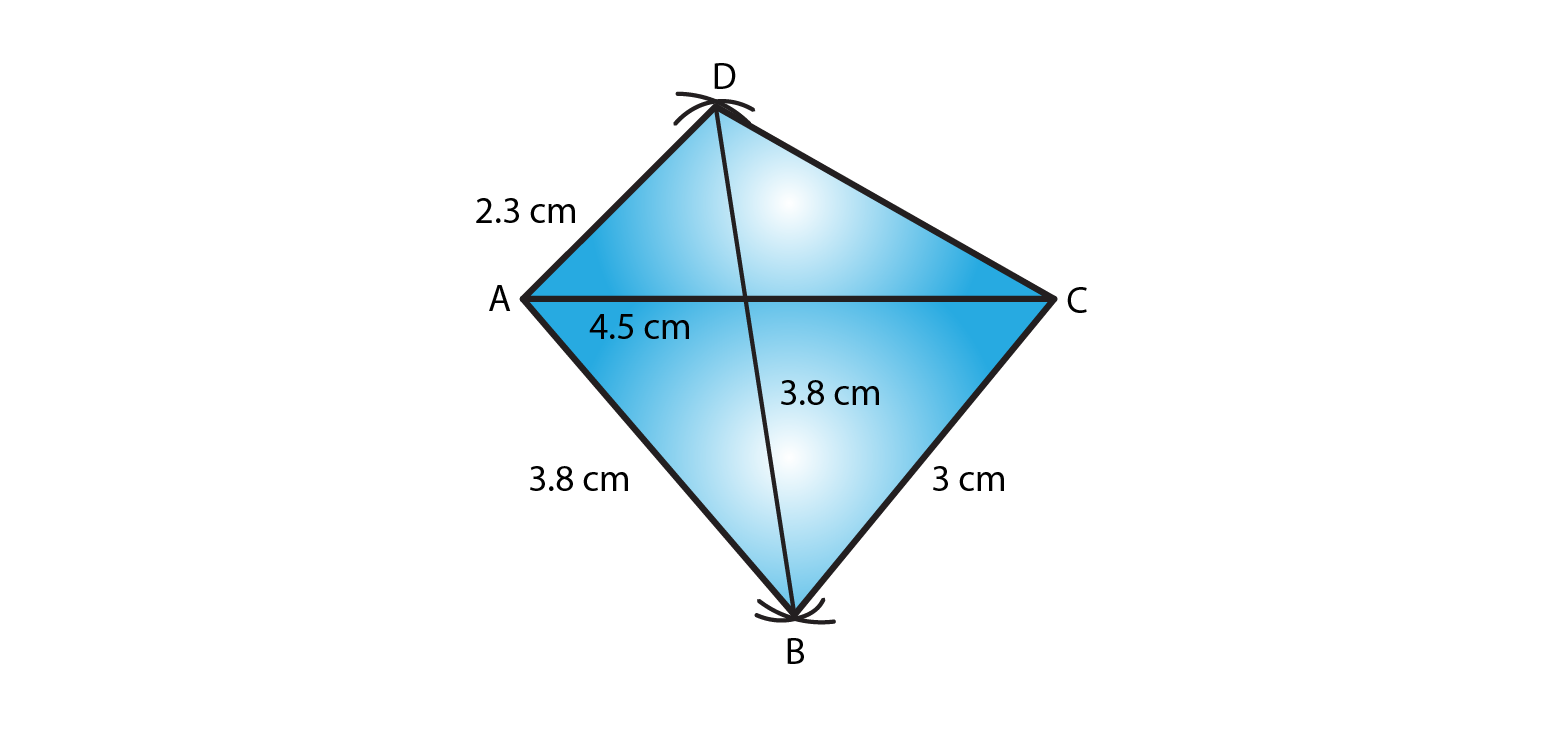2. Construct a quadrilateral ABCD in which BC = 7.5 cm, AC = AD = 6 cm, CD = 5 cm and BD = 10 cm.

Solution:

The given details are BC = 7.5 cm, AC = AD = 6 cm, CD = 5 cm and BD = 10 cm.

Step 1- Draw a line AC = 6cm.

Step 2- Cut an arc of radius 6cm with A as the center to mark that point as D.

Step 3- Cut an arc of radius 5cm with C as the center to intersect at point D.

Step 4- Cut an arc of radius 10cm with D as the center to mark that point as B.

Step 5- Cut an arc of radius 7.5cm with C as the center to intersect at point B.

Step 6- Now join AD, CD, DB and AB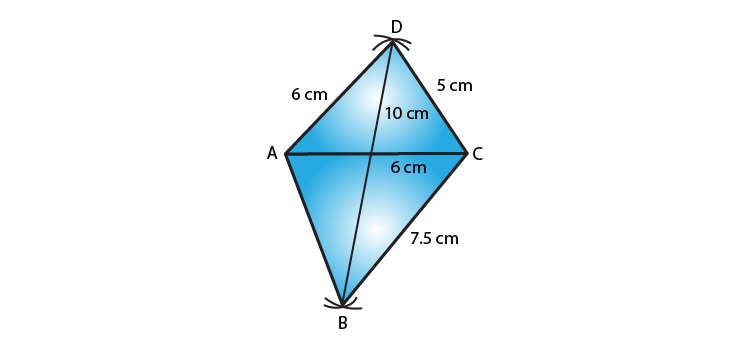3. Construct a quadrilateral ABCD when AB = 3 cm, CD = 3 cm, DA = 7.5 cm, AC = 8 cm and BD = 4 cm.

Solution:

The given details are AB = 3 cm, CD = 3 cm, DA = 7.5 cm, AC = 8 cm and BD = 4 cm.

Consider a triangle ABD from the given data,

So, AB + BD = 3+4 = 7cm

We know that sum of lengths of two sides of a triangle is always greater than the third side.

∴ The construction is not possible.

4. Construct a quadrilateral ABCD given AD = 3.5 cm, BC = 2.5 cm, CD = 4.1 cm, AC = 7.3 cm and BD = 3.2 cm.

Solution:

The given details are AD = 3.5 cm, BC = 2.5 cm, CD = 4.1 cm, AC = 7.3 cm and BD = 3.2 cm.

Step 1- Draw a line CD = 4.1cm

Step 2- Cut an arc of radius 7.3cm with C as the center to mark that point as A.

Step 3- Cut an arc of radius 3.5cm with D as the center to intersect at point A.

Step 4- Cut an arc of radius 3.2cm with D as the center to mark that point as B.

Step 5- Cut an arc of radius 2.5cm with C as the center to intersect at point B.

Step 6- Now join CA, DA, DB, CB and AB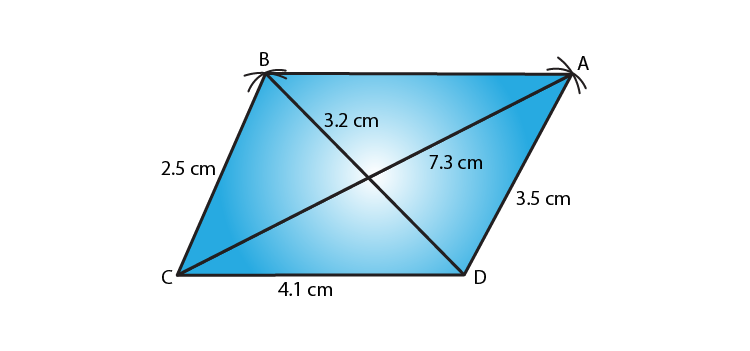5. Construct a quadrilateral ABCD given AD = 5 cm, AB = 5.5 cm, BC = 2.5 cm, AC = 7.1 cm and BD = 8 cm.

Solution:

The given details are AD = 5 cm, AB = 5.5 cm, BC = 2.5 cm, AC = 7.1 cm and BD = 8 cm.

Step 1- Draw a line AB = 5.5cm

Step 2- Cut an arc of radius 2.5cm with B as the center to mark that point as C.

Step 3- Cut an arc of radius 7.1cm with A as the center to intersect at point C.

Step 4- Cut an arc of radius 8cm with B as the center to mark that point as D.

Step 5- Cut an arc of radius 5cm with A as the center to intersect at point D.

Step 6- Now join BC, AC, BD, AD and CD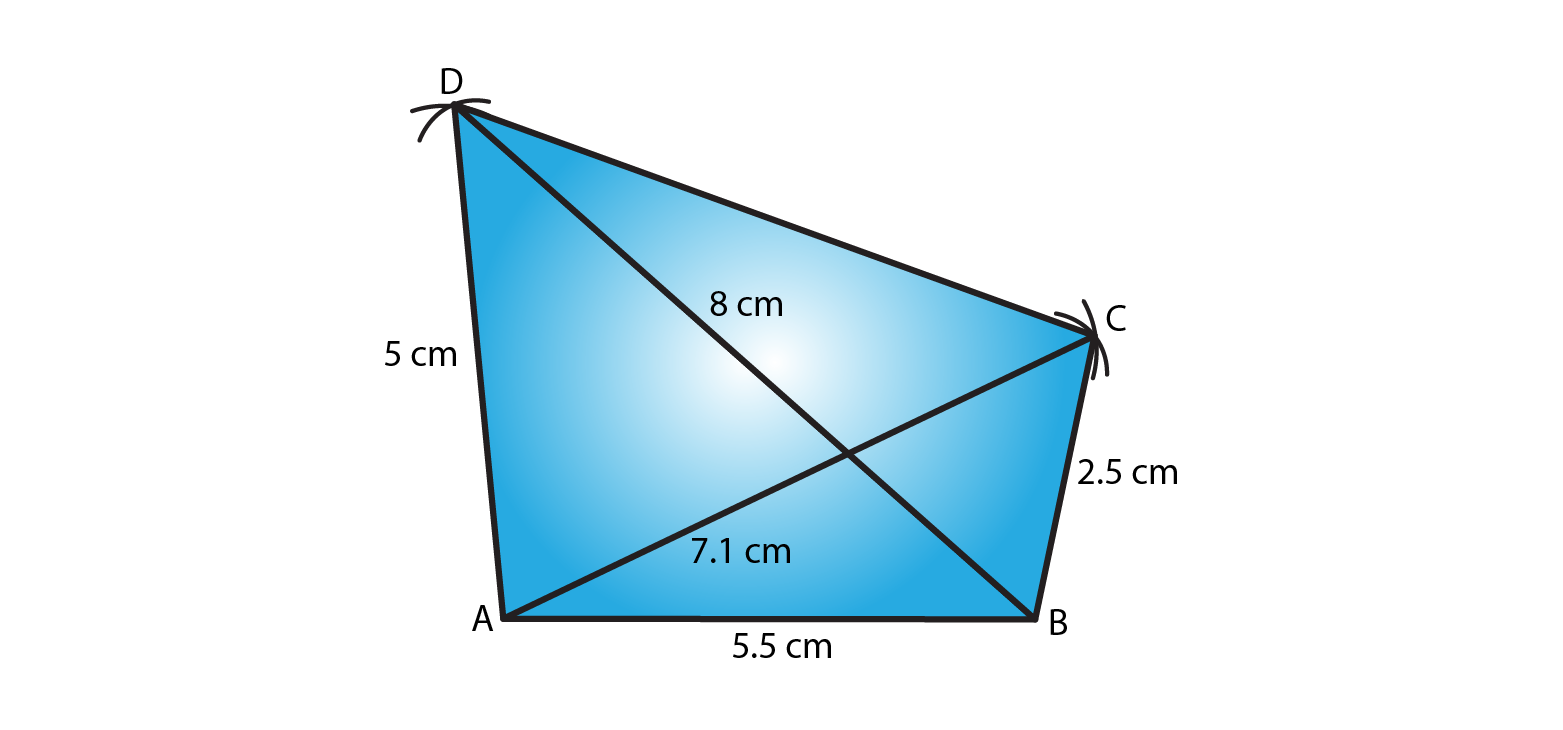6. Construct a quadrilateral ABCD in which BC = 4 cm, CA = 5.6 cm, AD = 4.5 cm, CD = 5 cm and BD = 6.5 cm.

Solution:

The given details are BC = 4 cm, CA = 5.6 cm, AD = 4.5 cm, CD = 5 cm and BD = 6.5 cm.

Step 1- Draw a line BC = 4cm

Step 2- Cut an arc of radius 6.5cm with B as the center to mark that point as D.

Step 3- Cut an arc of radius 5cm with C as the center to intersect at point D.

Step 4- Cut an arc of radius 5.6cm with C as the center to mark that point as A.

Step 5- Cut an arc of radius 4.5cm with D as the center to intersect at point A.

Step 6- Now join BD, CD, CA, DA and AB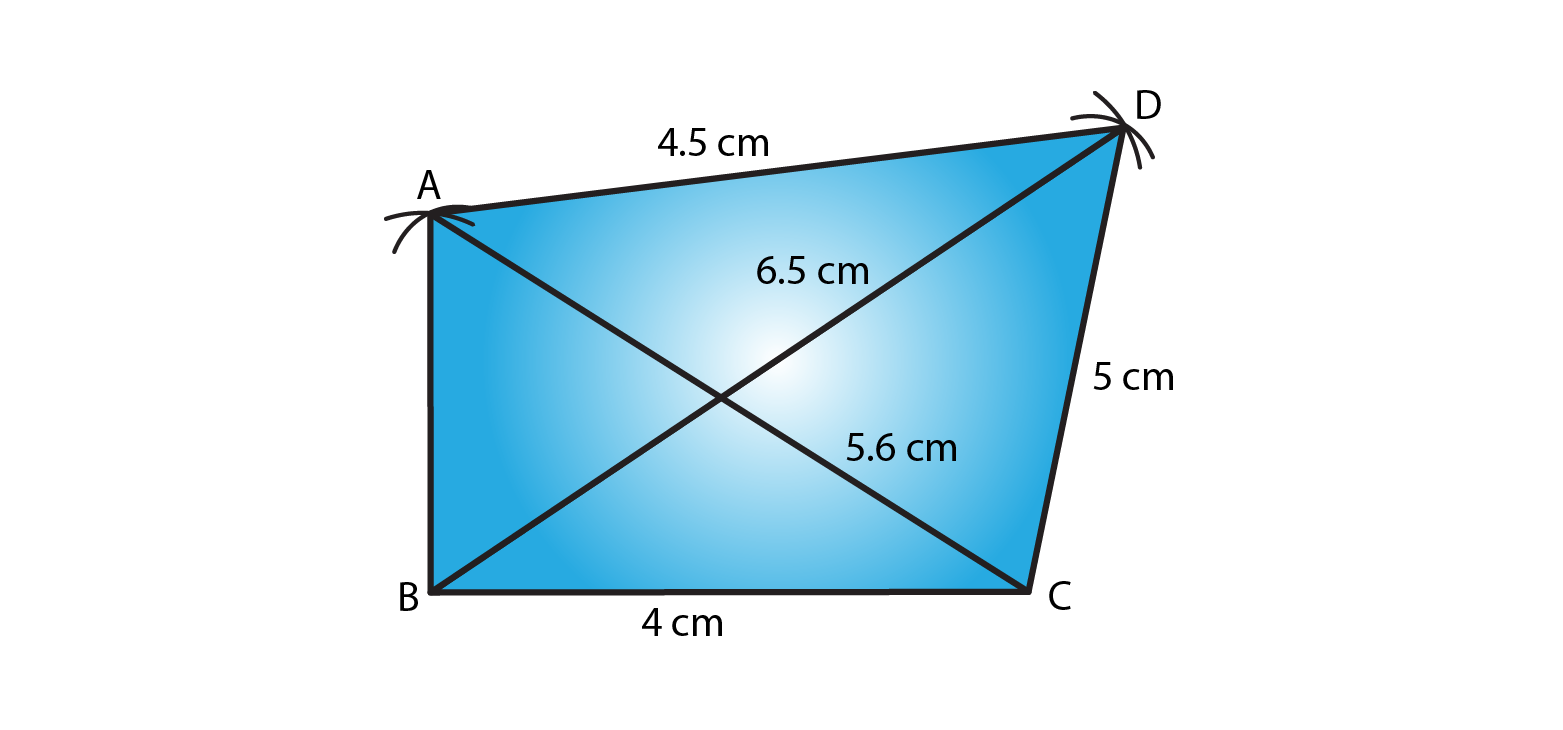EXERCISE 18.3 PAGE NO: 18.8

1. Construct a quadrilateral ABCD in which AB = 3.8 cm, BC = 3.4 cm, CD = 4.5 cm, AD = 5 cm and ∠B = 80°.

Solution:

The given details are AB = 3.8 cm, BC = 3.4 cm, CD = 4.5 cm, AD = 5 cm and ∠B = 80°.

Step 1- Draw a line AB = 3.8cm

Step 2- Construct and angle of 80o at B.

Step 3- Cut an arc of radius 3.4cm with B as the center to mark that point as C.

Step 4- Cut an arc of radius 5cm with A as the center to mark that point as D.

Step 5- Cut an arc of radius 4.5cm with C as the center to intersect at point D.

Step 6- Now join BC, AD and CD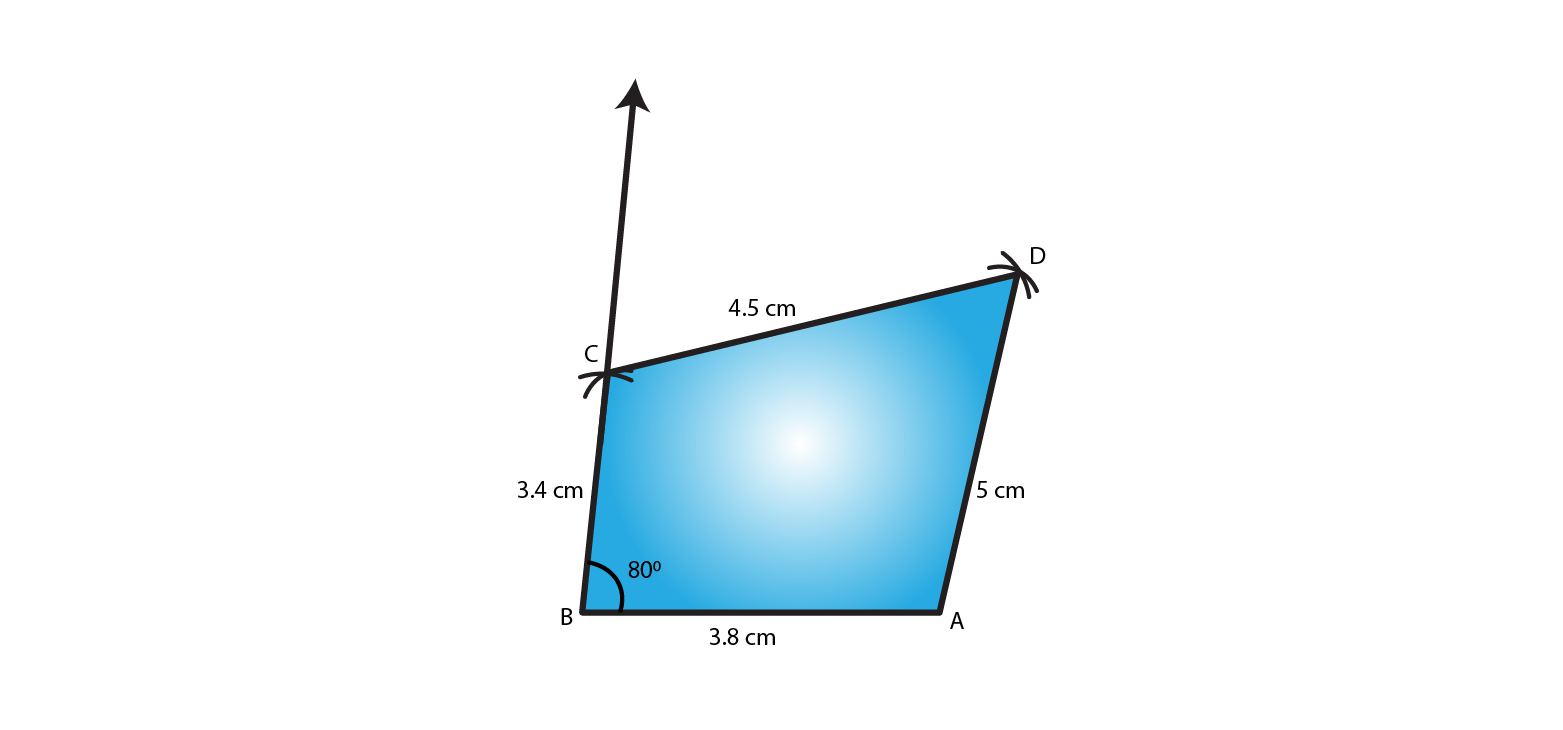2. Construct a quadrilateral ABCD given that AB = 8 cm, BC = 8 cm, CD = 10 cm, AD = 10 cm and ∠A = 45°.

Solution:

The given details are AB = 8 cm, BC = 8 cm, CD = 10 cm, AD = 10 cm and ∠A = 45°.

Step 1- Draw a line AB = 8cm

Step 2- Construct and angle of 45o at A.

Step 3- Cut an arc of radius 10cm with A as the center to mark that point as D.

Step 4- Cut an arc of radius 10cm with D as the center to mark that point as C.

Step 5- Cut an arc of radius 8cm with B as the center to intersect at point C.

Step 6- Now join AD, DC and BC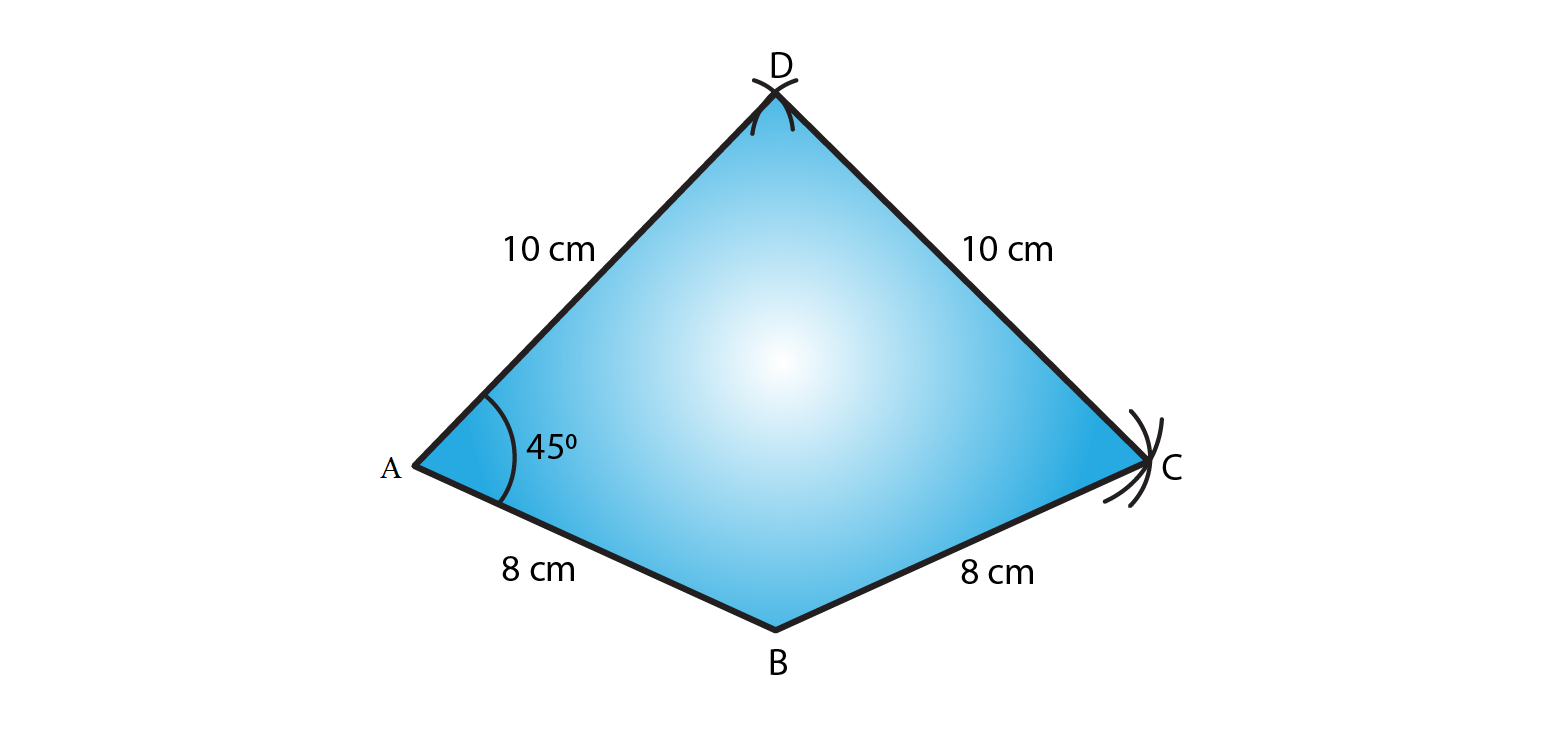3. Construct a quadrilateral ABCD in which AB = 7.7 cm, BC = 6.8 cm, CD = 5.1 cm, AS = 3.6 cm and ∠C = 120°.

Solution:

The given details are AB = 7.7 cm, BC = 6.8 cm, CD = 5.1 cm, AS = 3.6 cm and ∠C = 120°.

Step 1- Draw a line DC = 5.1cm

Step 2- Construct and angle of 120o at C.

Step 3- Cut an arc of radius 6.8cm with C as the center to mark that point as B.

Step 4- Cut an arc of radius 7.7cm with B as the center to mark that point as A.

Step 5- Cut an arc of radius 3.6cm with D as the center to intersect at point A.

Step 6- Now join CB, BA and DA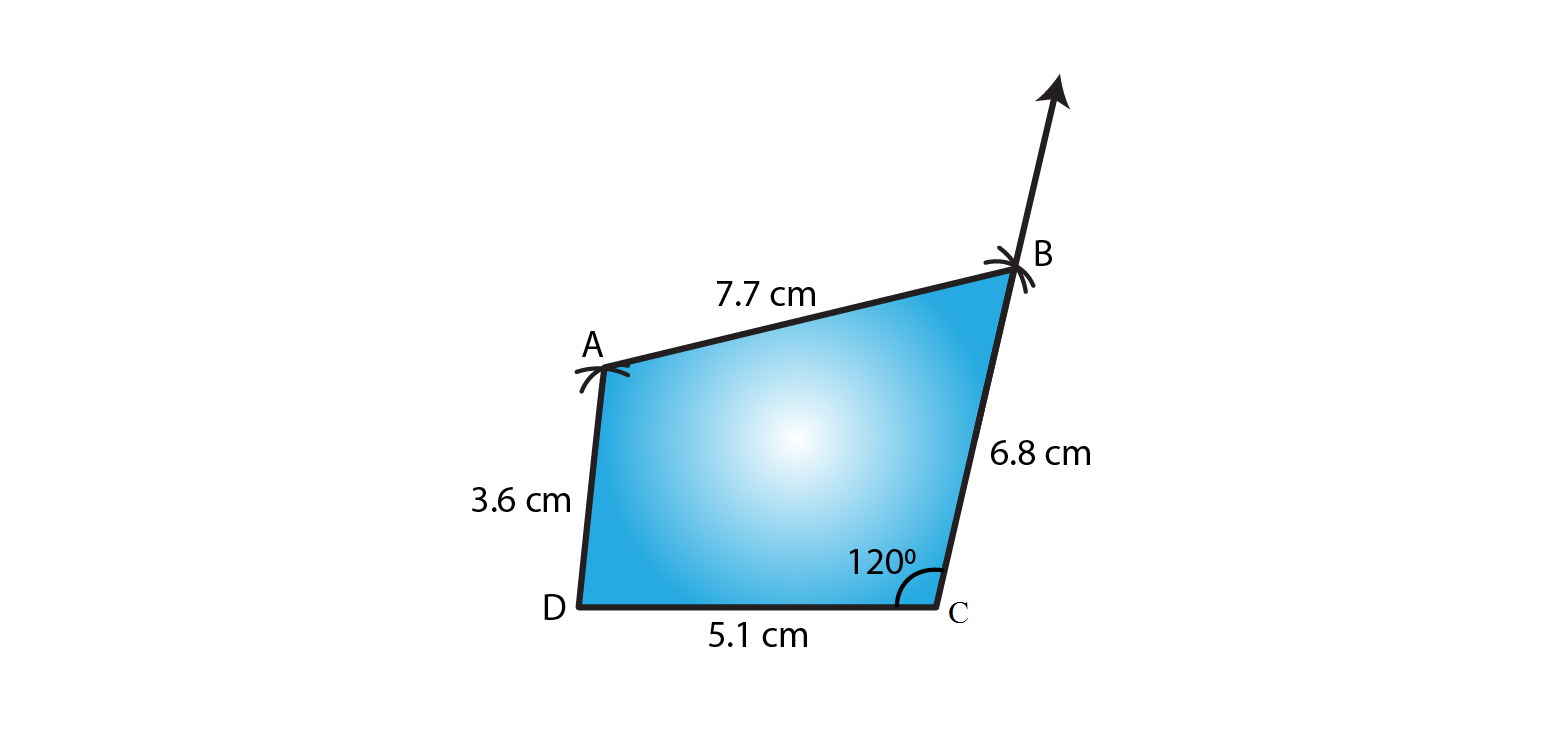4. Construct a quadrilateral ABCD in which AB = BC = 3 cm, AD = CD = 5 cm and ∠B = 120°.

Solution:

The given details are AB = BC = 3 cm, AD = CD = 5 cm and ∠B = 120°.

Step 1- Draw a line AB = 3cm

Step 2- Construct and angle of 120o at B.

Step 3- Cut an arc of radius 3cm with B as the center to mark that point as C.

Step 4- Cut an arc of radius 5cm with C as the center to mark that point as D.

Step 5- Cut an arc of radius 5cm with A as the center to intersect at point D.

Step 6- Now join BC, CD and DA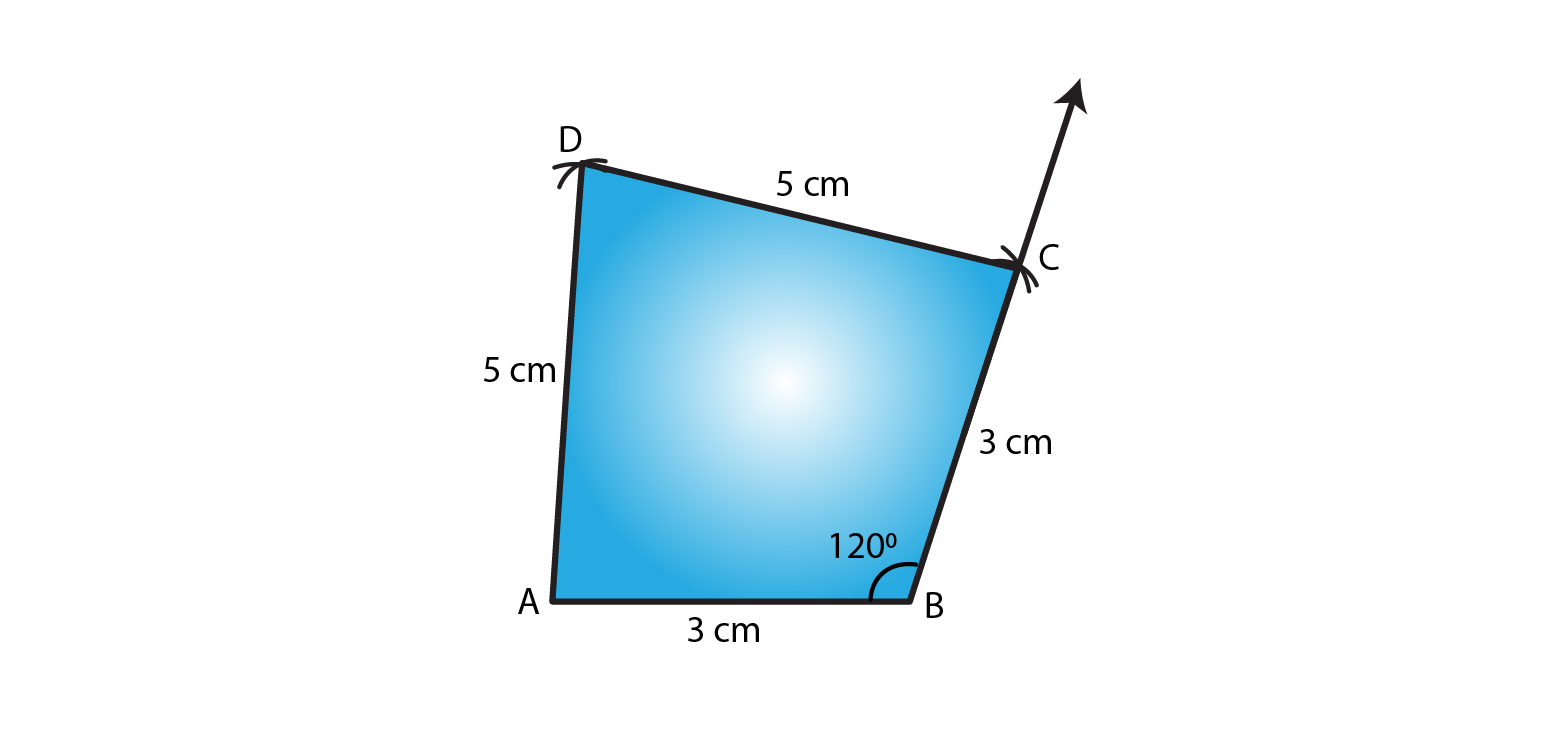5. Construct a quadrilateral ABCD in which AB = 2.8 cm, BC = 3.1 cm, CD = 2.6 cm and DA = 3.3 cm and ∠A = 60°.

Solution:

The given details are AB = 2.8 cm, BC = 3.1 cm, CD = 2.6 cm and DA = 3.3 cm and ∠A = 60°.

Step 1- Draw a line AB = 2.8cm

Step 2- Construct and angle of 60o at A.

Step 3- Cut an arc of radius 3.3cm with A as the center to mark that point as D.

Step 4- Cut an arc of radius 2.6cm with D as the center to mark that point as C.

Step 5- Cut an arc of radius 3.1cm with B as the center to intersect at point C.

Step 6- Now join AD, DC and CB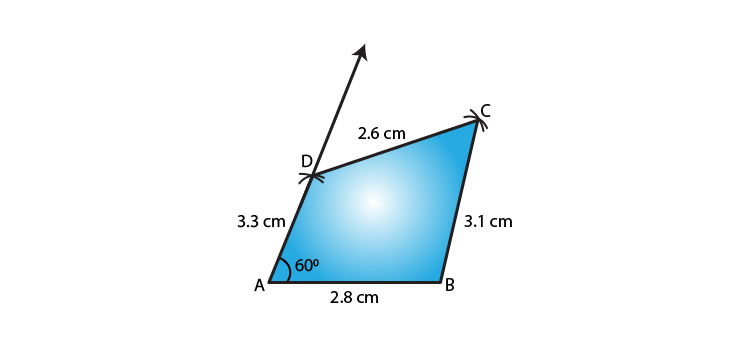6. Construct a quadrilateral ABCD in which AB = BC = 6 cm, AD = DC = 4.5 cm and ∠B = 120°.

Solution:

The given details are AB = BC = 6 cm, AD = DC = 4.5 cm and ∠B = 120°.

Step 1- Draw a line AB = 6cm

Step 2- Construct and angle of 120o at B.

Step 3- Cut an arc of radius 6cm with B as the center to mark that point as C.

Here, AC is about 10.3cm in length which is greater than AD + CD = 4.5+4.5=9cm

We know that sum of the two sides of a triangle is always greater than the third side.

∴ Construction is not possible.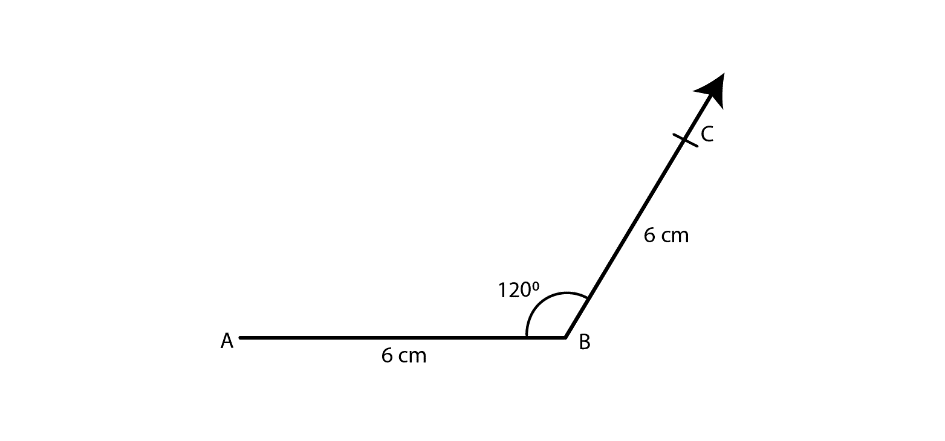EXERCISE 18.4 PAGE NO: 18.10

1. Construct a quadrilateral ABCD in which AB = 6 cm, BC = 4 cm, CD = 4 cm, ∠B = 95° and ∠C = 90°.

Solution:

The given details are AB = 6 cm, BC = 4 cm, CD = 4 cm, ∠B = 95° and ∠C = 90°.

Step 1- Draw a line BC = 4cm

Step 2- Construct and angle of 95o at B.

Step 3- Cut an arc of radius 6cm with B as the center to mark that point as A.

Step 4- Construct and angle of 90o at C.

Step 5- Cut an arc of radius 4cm with C as the center to mark that point as D.

Step 6- Now join BA, CD and AD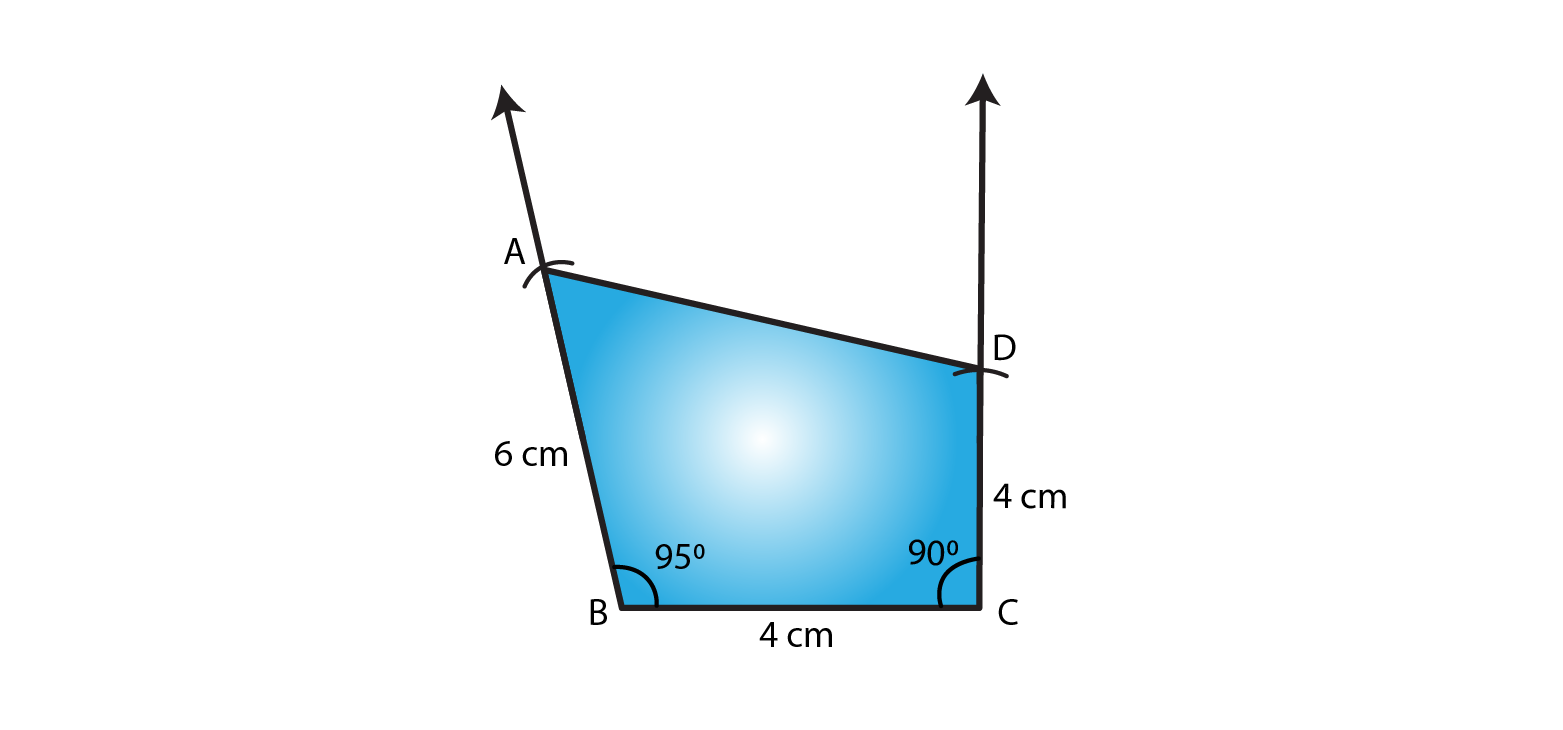2. Construct a quadrilateral ABCD where AB = 4.2cm, BC = 3.6 cm, CD = 4.8 cm, ∠B = 30° and ∠C = 150°.

Solution:

The given details are AB = 4.2cm, BC = 3.6 cm, CD = 4.8 cm, ∠B = 30° and ∠C = 150°.

Step 1- Draw a line BC = 3.6cm

Step 2- Construct and angle of 30o at B.

Step 3- Cut an arc of radius 4.2cm with B as the center to mark that point as A.

Step 4- Construct and angle of 150o at C.

Step 5- Cut an arc of radius 4.8cm with C as the center to mark that point as D.

Step 6- Now join BA, CD and AD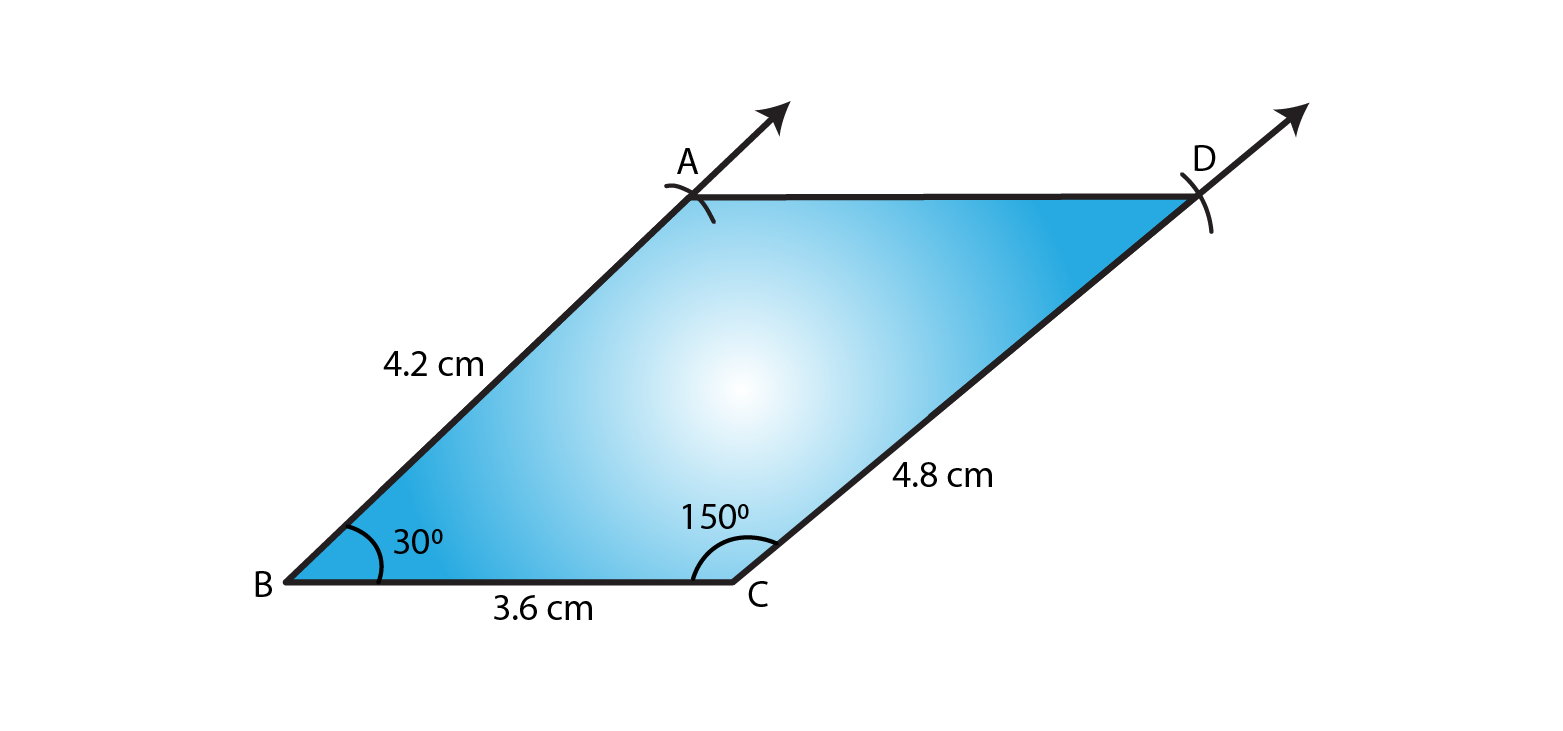3. Construct a quadrilateral PQRS in which PQ = 3.5 cm, QR = 2.5 cm, RS = 4.1 cm, ∠Q = 75° and ∠R = 120°.

Solution:

The given details are PQ = 3.5 cm, QR = 2.5 cm, RS = 4.1 cm, ∠Q = 75° and ∠R = 120°.

Step 1- Draw a line QR = 2.5cm

Step 2- Construct and angle of 75o at Q.

Step 3- Cut an arc of radius 3.5cm with Q as the center to mark that point as P.

Step 4- Construct and angle of 120o at R.

Step 5- Cut an arc of radius 4.1cm with R as the center to mark that point as S.

Step 6- Now join QP, RS and PS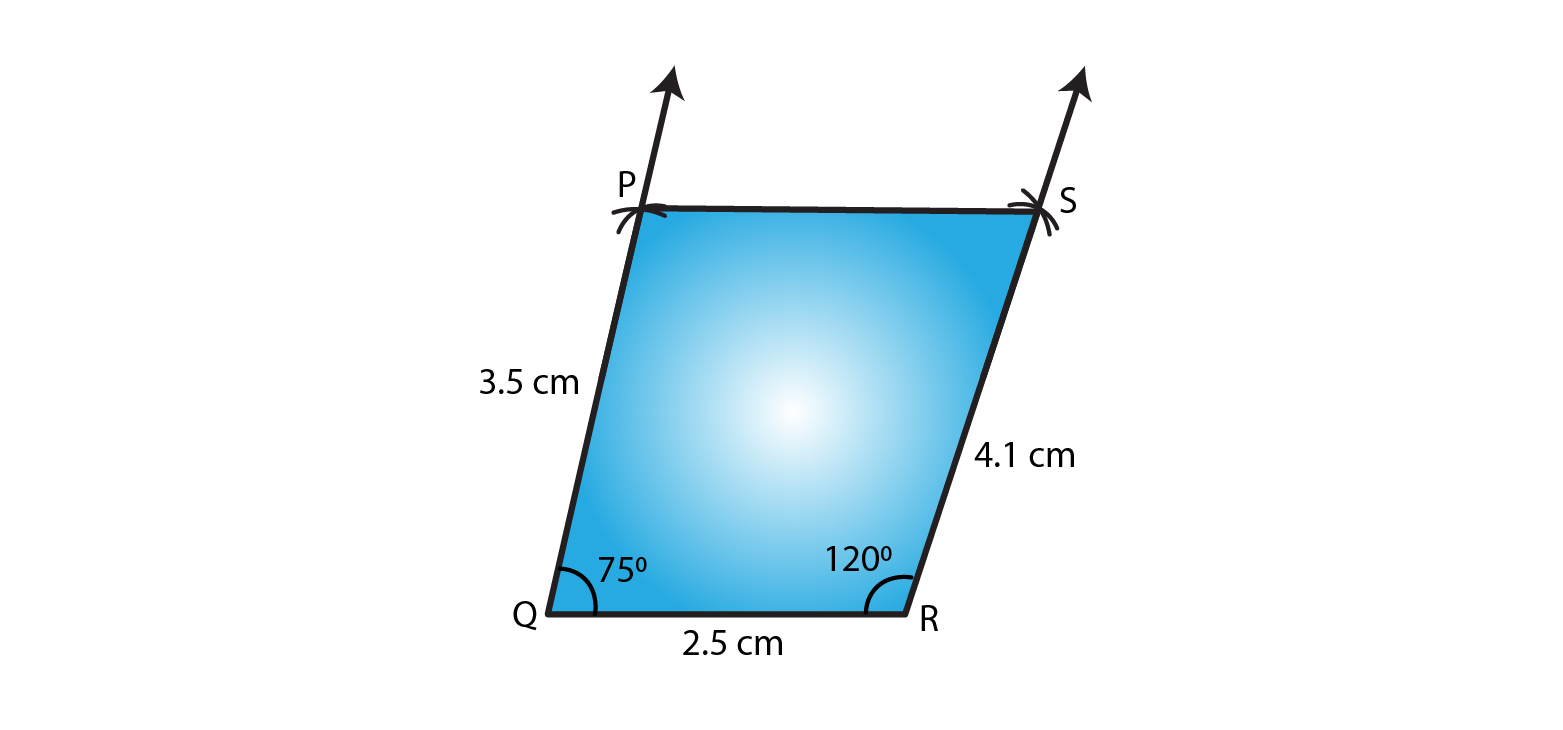4. Construct a quadrilateral ABCD given BC = 6.6 cm, CD = 4.4 cm, AD = 5.6 cm ∠D = 100° and ∠C = 95

Solution:

The given details are BC = 6.6 cm, CD = 4.4 cm, AD = 5.6 cm ∠D = 100° and ∠C = 95

Step 1- Draw a line DC = 4.4cm

Step 2- Construct and angle of 100o at D.

Step 3- Cut an arc of radius 5.6cm with D as the center to mark that point as A.

Step 4- Construct and angle of 95o at C.

Step 5- Cut an arc of radius 6.6cm with C as the center to mark that point as B.

Step 6- Now join DA, CB and AB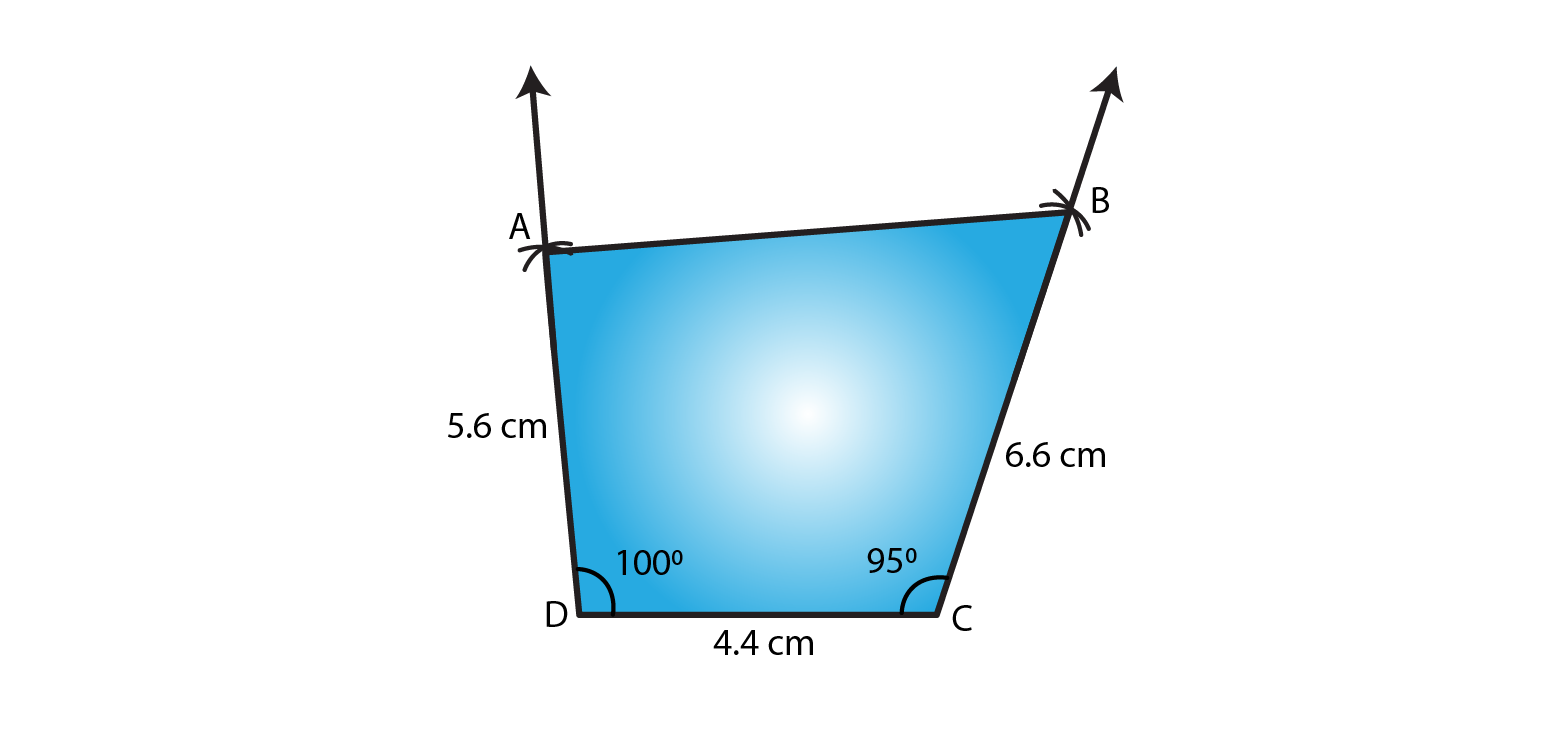5. Construct a quadrilateral ABCD in which AD = 3.5 cm, AB = 4.4 cm, BC = 4.7 cm, ∠A = 125° and ∠B = 120°.

Solution:

The given details are AD = 3.5 cm, AB = 4.4 cm, BC = 4.7 cm, ∠A = 125° and ∠B = 120°.

Step 1- Draw a line AB = 4.4cm

Step 2- Construct and angle of 125o at A.

Step 3- Cut an arc of radius 3.5cm with A as the center to mark that point as D.

Step 4- Construct and angle of 120o at B.

Step 5- Cut an arc of radius 4.7cm with B as the center to mark that point as C.

Step 6- Now join AD, BC and CD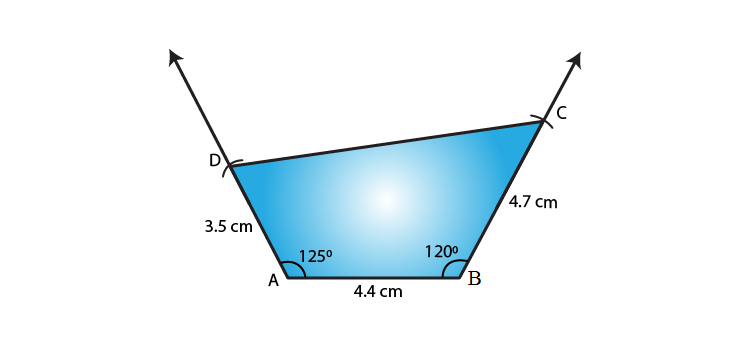6. Construct a quadrilateral PQRS in which ∠Q = 45° and ∠R = 90°, QR = 5 cm, PQ = 9 cm and RS = 7 cm.

Solution:

The given details are ∠Q = 45° and ∠R = 90°, QR = 5 cm, PQ = 9 cm and RS = 7 cm.

Step 1- Draw a line QR = 5cm

Step 2- Construct and angle of 45o at Q.

Step 3- Cut an arc of radius 9cm with Q as the center to mark that point as P.

Step 4- Construct and angle of 90o at R.

Step 5- Cut an arc of radius 7cm with R as the center to mark that point as S.

Step 6- Now join QP, RS

Since the line segment QP and RS are not intersecting at each other, quadrilateral cannot be formed.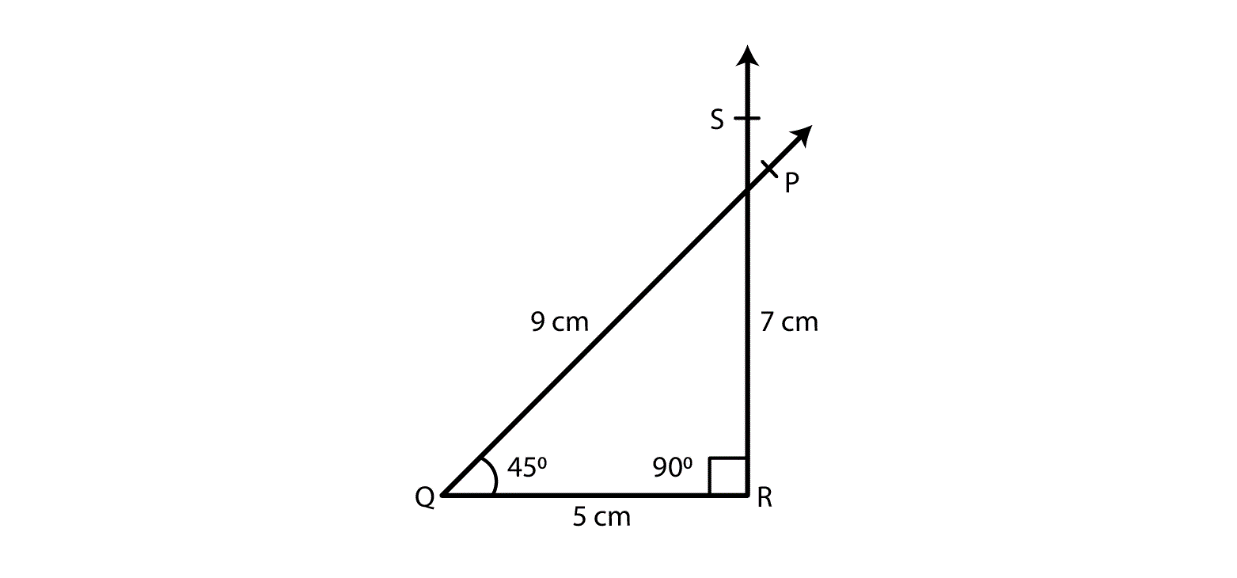7. Construct a quadrilateral ABCD in which AB = BC = 3 cm, AD = 5 cm, ∠A = 90° and ∠B = 105°.

Solution:

The given details are AB = BC = 3 cm, AD = 5 cm, ∠A = 90° and ∠B = 105°.

Step 1- Draw a line AB = 3cm

Step 2- Construct and angle of 90o at A.

Step 3- Cut an arc of radius 5cm with A as the center to mark that point as D.

Step 4- Construct and angle of 105o at B.

Step 5- Cut an arc of radius 3cm with B as the center to mark that point as C.

Step 6- Now join AD, BC and CD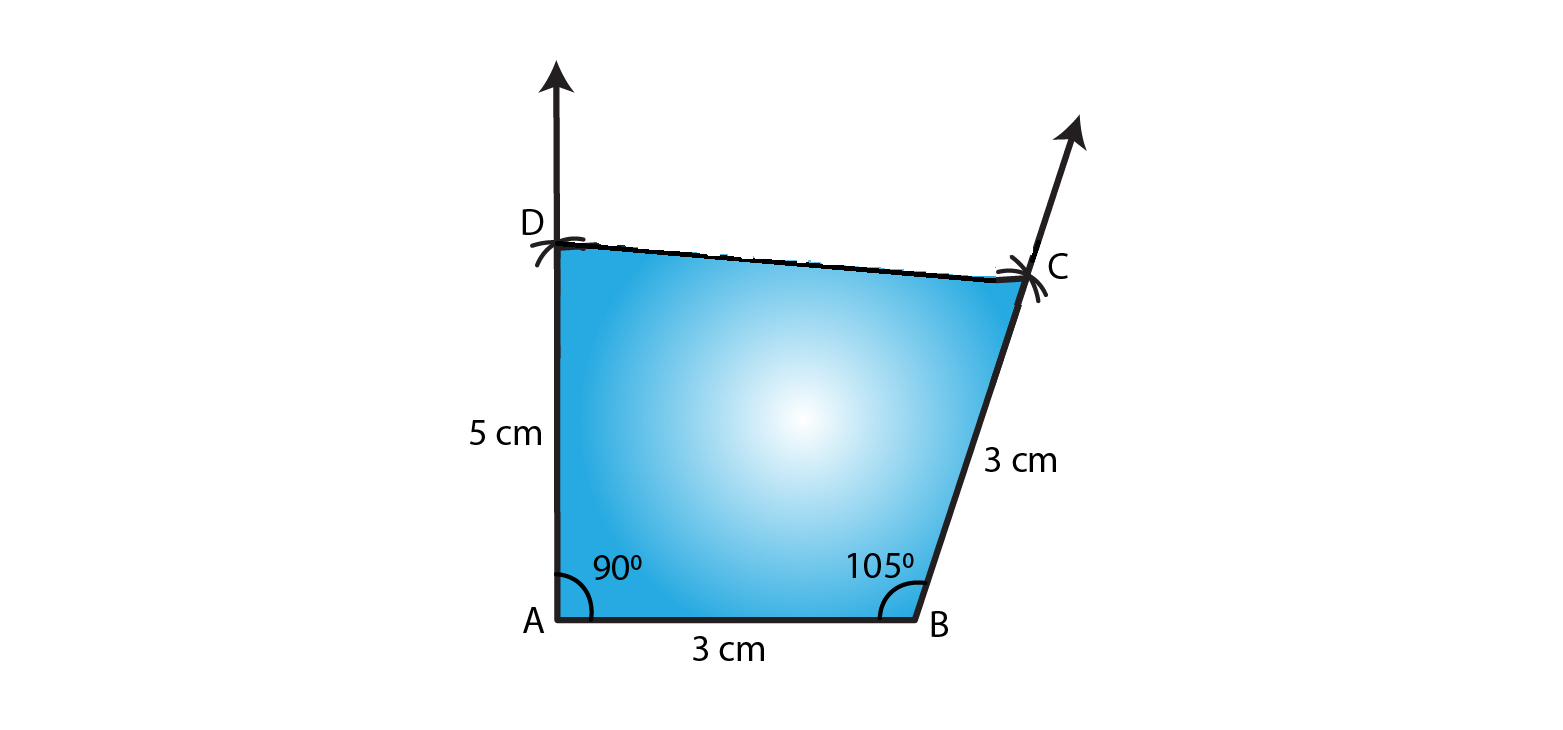8. Construct a quadrilateral BDEF, where DE = 4.5 cm, EF = 3.5 cm, FB = 6.5 cm, ∠F = 50° and ∠E = 100°.

Solution:

The given details are DE = 4.5 cm, EF = 3.5 cm, FB = 6.5 cm, ∠F = 50° and ∠E = 100°.

Step 1- Draw a line EF = 3.5cm

Step 2- Construct and angle of 100o at E.

Step 3- Cut an arc of radius 4.5cm with E as the center to mark that point as D.

Step 4- Construct and angle of 50o at F.

Step 5- Cut an arc of radius 6.5cm with F as the center to mark that point as B.

Step 6- Now join DE, FB and DB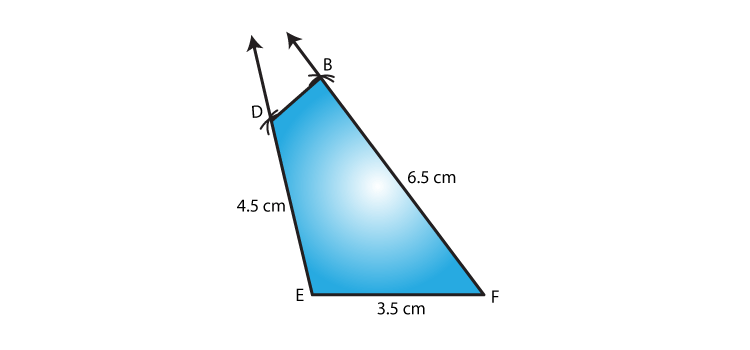EXERCISE 18.5 PAGE NO: 18.13

1. Construct a quadrilateral ABCD given that AB = 4 cm, BC = 3 cm, ∠A = 75°, ∠B = 80° and ∠C = 120°.

Solution:

The given details are AB = 4 cm, BC = 3 cm, ∠A = 75°, ∠B = 80° and ∠C = 120°.

Step 1- Draw a line AB = 4cm

Step 2- Construct and angle of 75o at A.

Step 3- Construct and angle of 80o at B.

Step 4- Cut an arc of radius 3cm with B as the center to mark that point as C.

Step 5- Construct and angle of 120o at C such that it meets the line segment AX, mark that point as D.

Step 6- Now join BC, CD and DA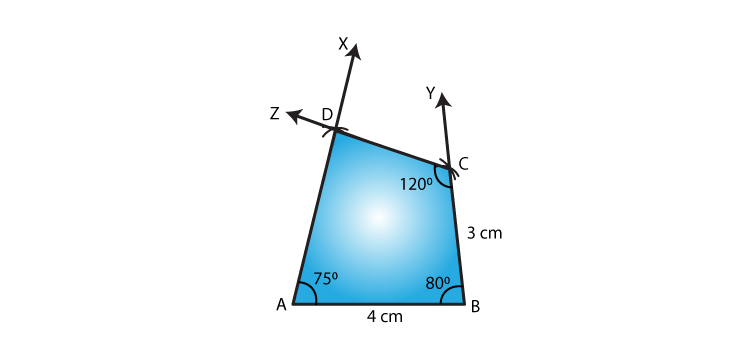2. Construct a quadrilateral ABCD where AB = 5.5 cm, BC = 3.7 cm, ∠A = 60°, ∠B = 105° and ∠D = 90°.

Solution:

The given details are AB = 5.5 cm, BC = 3.7 cm, ∠A = 60°, ∠B = 105° and ∠D = 90°.

We know that ∠A + ∠B + ∠C + ∠D = 360o

∴ ∠C = 105o

Step 1- Draw a line AB = 5.5cm

Step 2- Construct and angle of 60o at A.

Step 3- Construct and angle of 105o at B.

Step 4- Cut an arc of radius 3.7cm with B as the center to mark that point as C.

Step 5- Construct and angle of 105o at C such that it meets the line segment AX, mark that point as D.

Step 6- Now join BC, CD and DA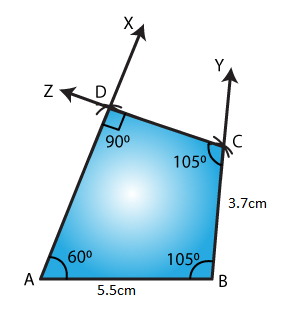3. Construct a quadrilateral PQRS where PQ = 3.5 cm, QR = 6.5 cm, ∠P = ∠R = 105° and ∠S = 75°.

Solution:

The given details are PQ = 3.5 cm, QR = 6.5 cm, ∠P = ∠R = 105° and ∠S = 75°.

We know that ∠P + ∠Q + ∠R + ∠S = 360o

∴ ∠Q = 75o

Step 1- Draw a line PQ = 3.5cm

Step 2- Construct and angle of 105o at P.

Step 3- Construct and angle of 75o at Q.

Step 4- Cut an arc of radius 6.5cm with Q as the center to mark that point as R.

Step 5- Construct and angle of 105o at R such that it meets the line segment PX, mark that point as S.

Step 6- Now join QR, RS and PS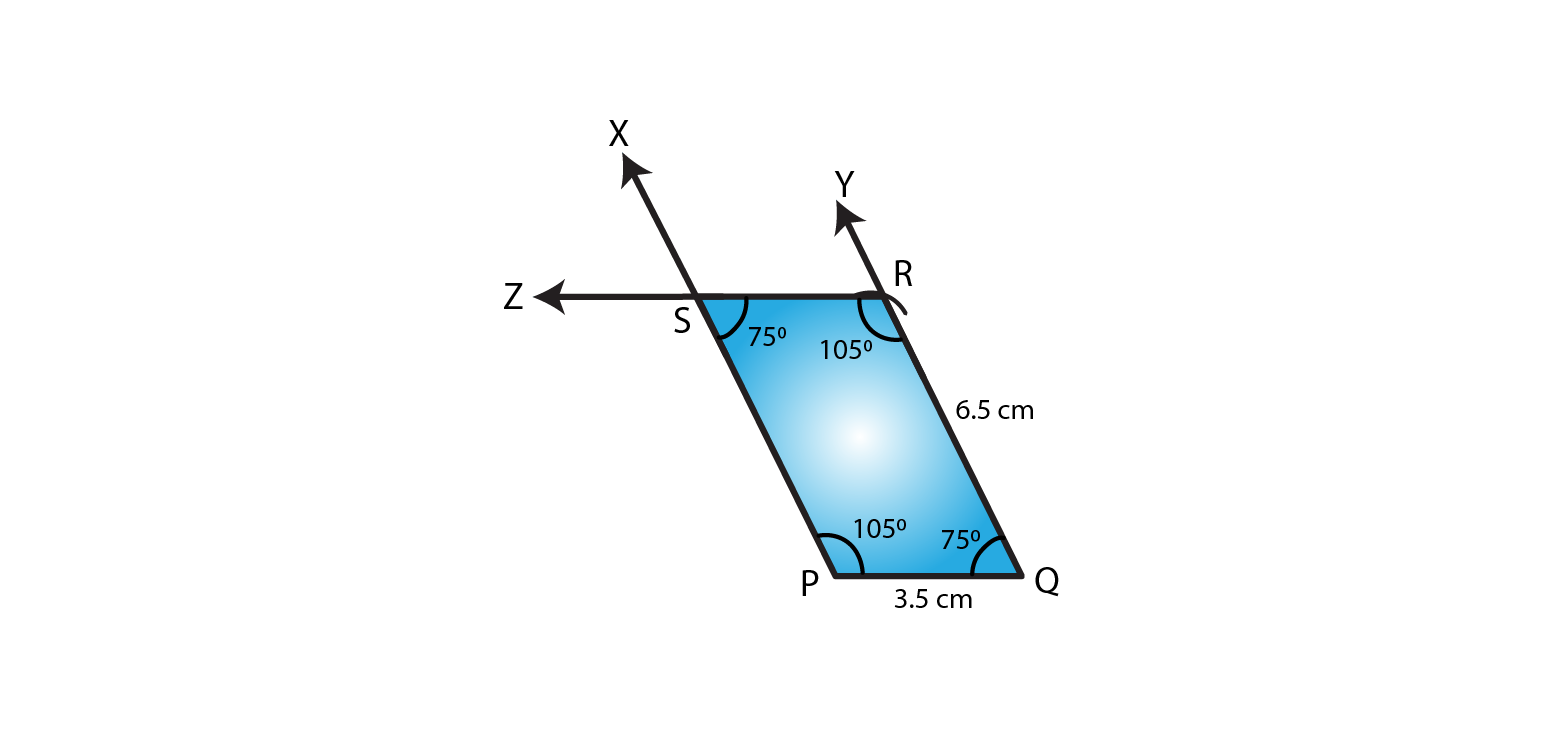4. Construct a quadrilateral ABCD when BC = 5.5 cm, CD = 4.1 cm, ∠A = 70°, ∠B = 110° and ∠D = 85°.

Solution:

The given details are BC = 5.5 cm, CD = 4.1 cm, ∠A = 70°, ∠B = 110° and ∠D = 85°.

We know that ∠A + ∠B + ∠C + ∠D = 360o

∴ ∠C = 95o

Step 1- Draw a line BC = 5.5cm

Step 2- Construct and angle of 110o at B.

Step 3- Construct and angle of 95o at C.

Step 4- Cut an arc of radius 4.1cm with C as the center to mark that point as D.

Step 5- Construct and angle of 85o at D such that it meets the line segment BX, mark that point as A.

Step 6- Now join CD, DA and BA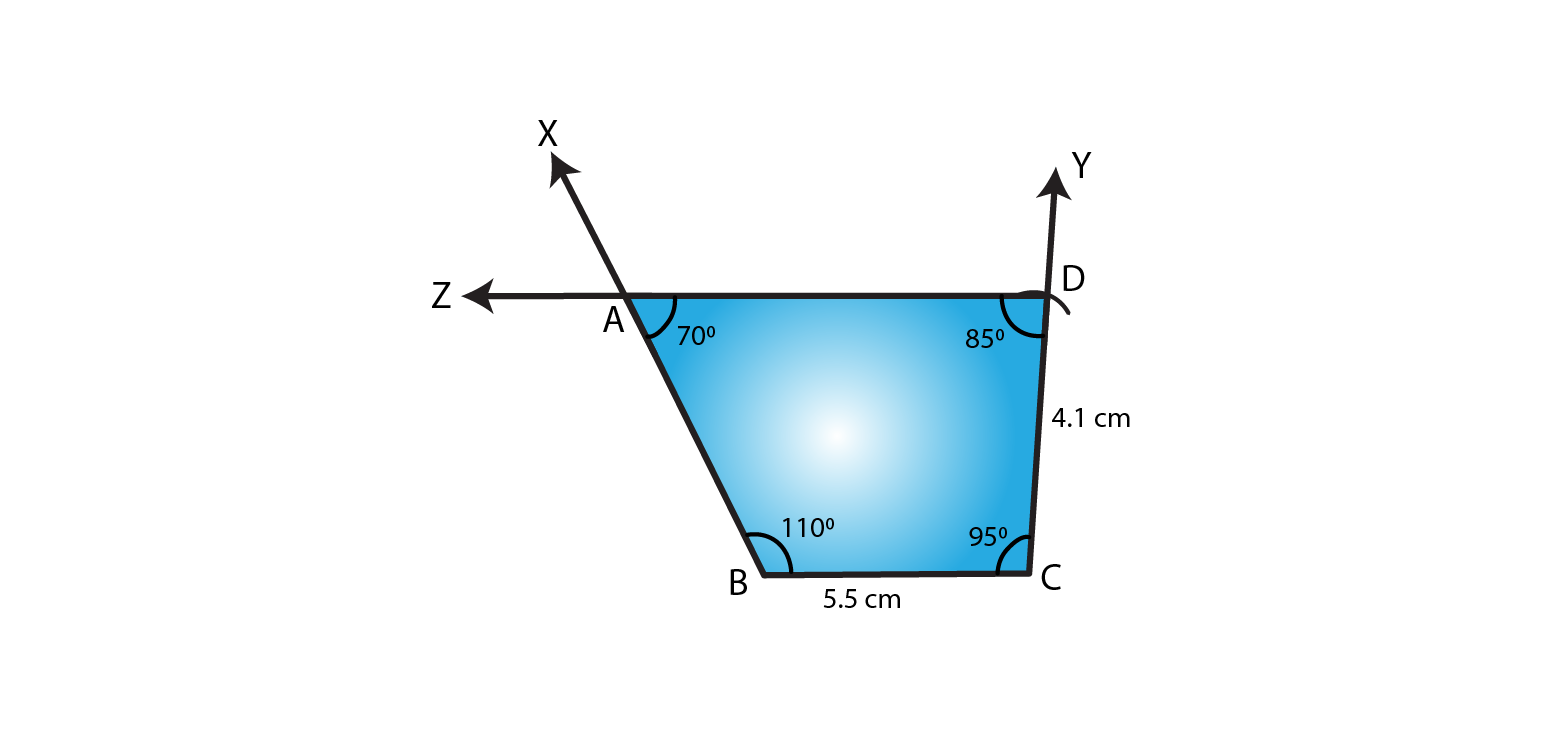5. Construct a quadrilateral ABCD ∠A = 65°, ∠B = 105°, ∠C = 75°, BC = 5.7 cm and CD = 6.8 cm.

Solution:

The given details are ∠A = 65°, ∠B = 105°, ∠C = 75°, BC = 5.7 cm and CD = 6.8 cm.

We know that ∠A + ∠B + ∠C + ∠D = 360o

∴ ∠D = 115o

Step 1- Draw a line BC = 5.7cm

Step 2- Construct and angle of 105o at B.

Step 3- Construct and angle of 75o at C.

Step 4- Cut an arc of radius 6.8cm with C as the center to mark that point as D.

Step 5- Construct and angle of 115o at D such that it meets the line segment BX, mark that point as A.

Step 6- Now join CD, DA and BA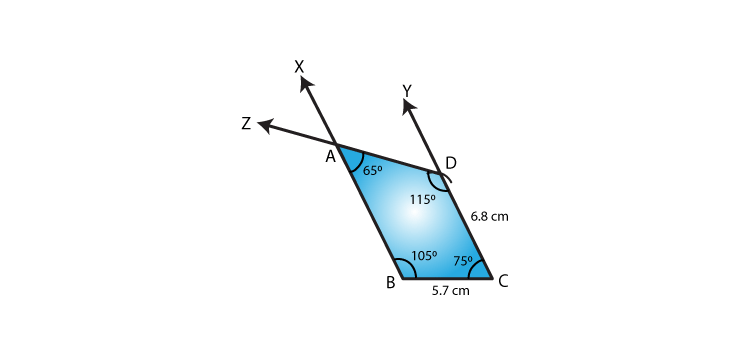6. Construct a quadrilateral PQRS in which PQ = 4 cm, QR = 5 cm ∠P = 50°, ∠Q = 110° and ∠R = 70°.

Solution:

The given details are PQ = 4 cm, QR = 5 cm ∠P = 50°, ∠Q = 110° and ∠R = 70°.

Step 1- Draw a line PQ = 4cm

Step 2- Construct and angle of 50o at P.

Step 3- Construct and angle of 110o at Q.

Step 4- Cut an arc of radius 5cm with Q as the center to mark that point as R.

Step 5- Construct and angle of 70o at R such that it meets the line segment PX, mark that point as S.

Step 6- Now join QR, RS and PS